# Introduction

This is an intermediate-level course that teaches you how to use Python for creating charts, plots, animations, and maps.View the Presentation ↗

# Notebooks and Datasets

This course uses Google Colab for all exercises. You do not need to install any packages or download any datasets.

The notebooks can be accessed by clicking on thebuttons at the beginning of each section. Once you have opened the notebook in Colab, you can copy it to your own account by going to File → Save a Copy in Drive.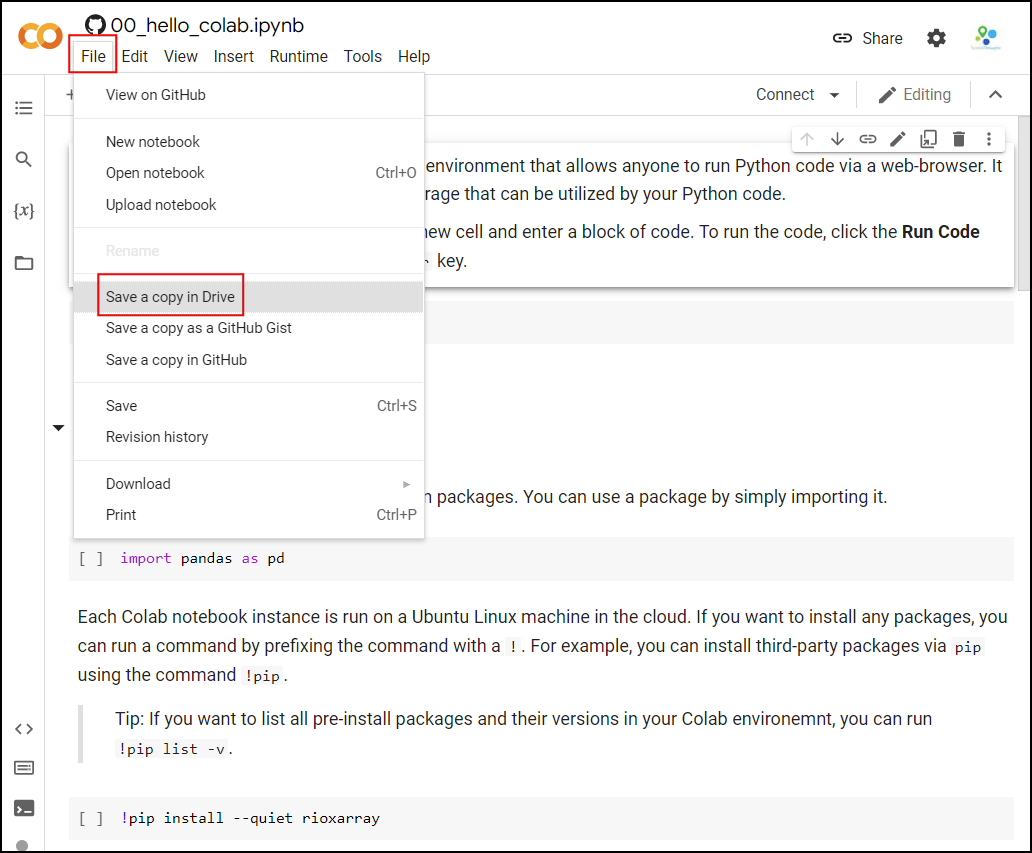Once the notebooks are saved to your drive, you will be able to modify the code and save the updated copy. You can also click the Share button and share a link to the notebook with others.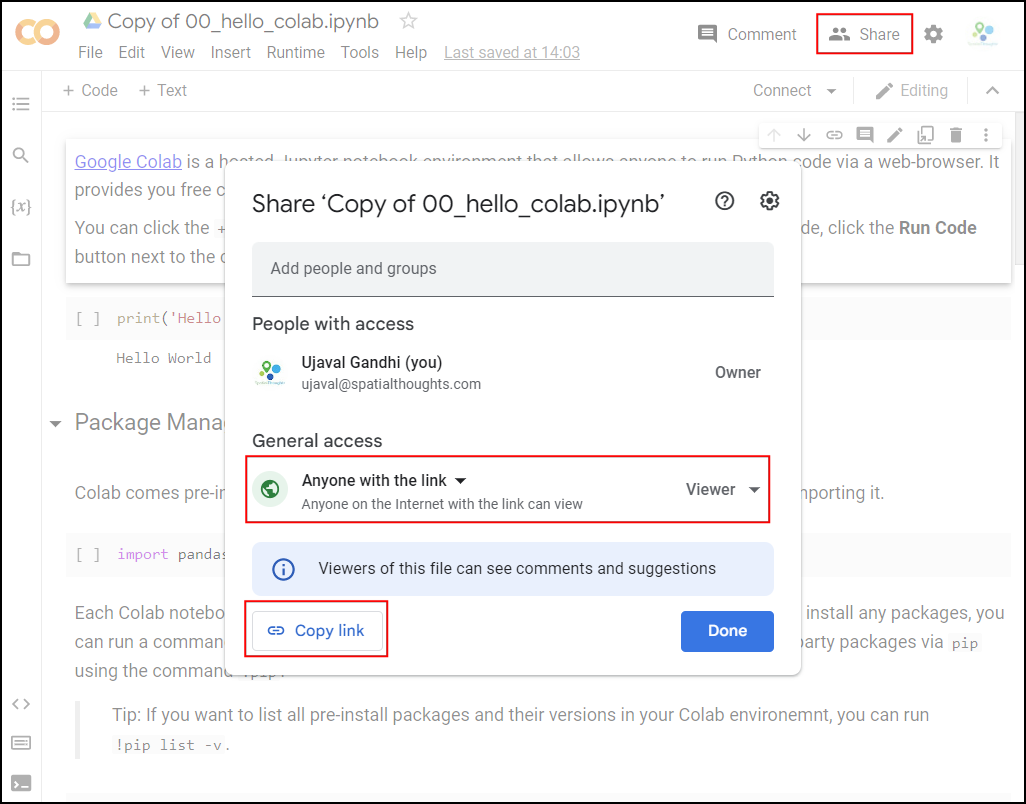# Hello ColabGoogle Colab is a hosted Jupyter notebook environment that allows anyone to run Python code via a web-browser. It provides you free computation and data storage that can be utilized by your Python code.

You can click the +Code button to create a new cell and enter a block of code. To run the code, click the Run Code button next to the cell, or press Shirt+Enter key.

print('Hello World')

### Package Management

Colab comes pre-installed with many Python packages. You can use a package by simply importing it.

import pandas as pd

Each Colab notebook instance is run on a Ubuntu Linux machine in the cloud. If you want to install any packages, you can run a command by prefixing the command with a !. For example, you can install third-party packages via pip using the command !pip.

Tip: If you want to list all pre-install packages and their versions in your Colab environemnt, you can run !pip list -v.

!pip install --quiet rioxarray
import rioxarray

Some packages may also require additional binaries or local configuration. This can be achieved using package management commands for Ubuntu Linux. For example, we can run apt command to install specific package required for geopandas to work correctly.

!apt install -qq libspatialindex-dev
!pip install --quiet fiona shapely pyproj rtree
!pip install --quiet geopandas
libspatialindex-dev is already the newest version (1.8.5-5).
The following package was automatically installed and is no longer required:
libnvidia-common-460
Use 'apt autoremove' to remove it.
0 upgraded, 0 newly installed, 0 to remove and 20 not upgraded.
import geopandas as gpd

### Data Management

Colab provides 100GB of disk space along with your notebook. This can be used to store your data, intermediate outputs and results.

The code below will create 2 folders named ‘data’ and ‘output’ in your local filesystem.

import os

data_folder = 'data'
output_folder = 'output'

if not os.path.exists(data_folder):
os.mkdir(data_folder)
if not os.path.exists(output_folder):
os.mkdir(output_folder)

We can download some data from the internet and store it in the Colab environment. Below is a helper function that uses urllib to fetch any file from a URL.

def download(url):
filename = os.path.join(data_folder, os.path.basename(url))
if not os.path.exists(filename):
from urllib.request import urlretrieve
local, _ = urlretrieve(url, filename)
print('Downloaded ' + local)

download('https://naciscdn.org/naturalearth/10m/cultural/' +
'ne_10m_populated_places_simple.zip')
Downloaded data/ne_10m_populated_places_simple.zip

The file is now in our local filesystem. We can construct the path to the data folder and read it using geopandas

file = 'ne_10m_populated_places_simple.zip'
filepath = os.path.join(data_folder, file)
places = gpd.read_file(filepath)

Let’s do some data processing and write the results to a new file. The code below will filter all places which are also country capitals.

capitals = places[places['adm0cap'] == 1]
capitals

We can write the results to the disk as a GeoPackage file.

output_file = 'capitals.gpkg'
output_path = os.path.join(output_folder, output_file)
capitals.to_file(driver='GPKG', filename=output_path)

You can open the Files tab from the left-hand panel in Colab and browse to the output folder. Locate the capitals.gpkg file and click the button and select Download to download the file locally.

# Matplotlib BasicsThis notebook introduces the Matplotlib library. This is one of the core Python packages for data visualization and is used by many spatial and non-spatial packages to create charts and maps.

## Setup

Most of the Matplotlib functionality is available in the pyplot submodule, and by convention is imported as plt

import os
import matplotlib.pyplot as plt
data_folder = 'data'
output_folder = 'output'

if not os.path.exists(data_folder):
os.mkdir(data_folder)
if not os.path.exists(output_folder):
os.mkdir(output_folder)

## Concepts

It is important to understand the 2 matplotlib objects

• Figure: This is the main container of the plot. A figure can contain multiple plots inside it
• Axes: Axes refers to an individual plot or graph. A figure contains 1 or more axes.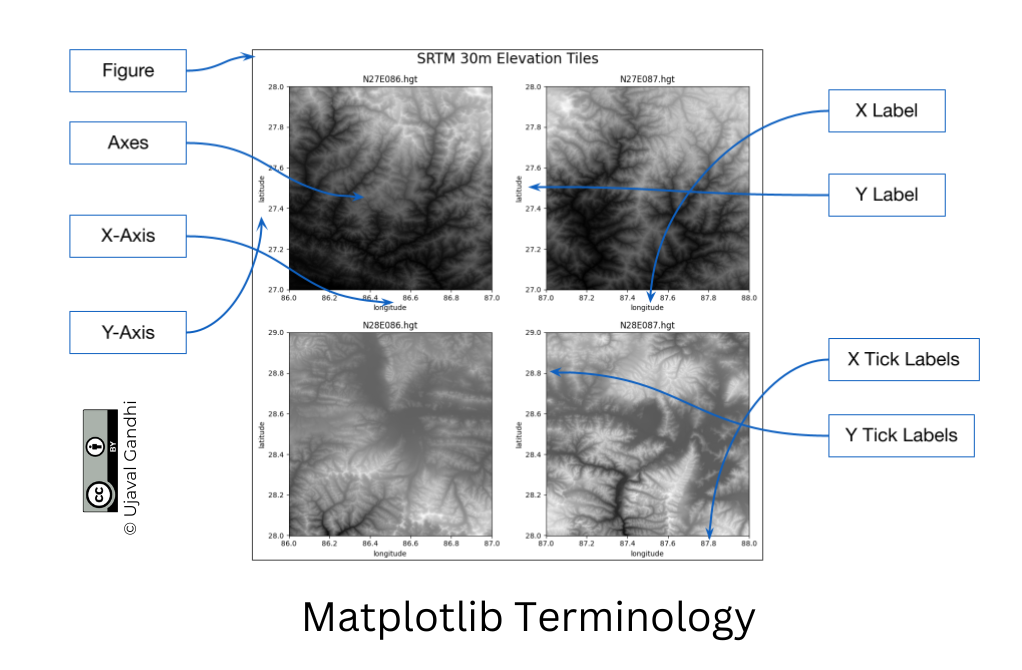We create a figure and a single subplot. Specifying 1 row and 1 column for the subplots() function create a figure and an axes within it. Even if we have a single plot in the figure, it is useful to use this logic of intialization so it is consistent across different scripts.

fig, ax = plt.subplots(1, 1)
fig.set_size_inches(5,5)
plt.show()

First, let’s learn how to plot a single point using matplotlib. Let’s say we want to display a point at the coordinate (0.5, 0.5).

point = (0.5, 0.5)

We display the point using the plot() function. The plot() function expects at least 2 arguments, first one being one or more x coordinates and the second one being one or more y coordinates. Remember that once a plot is displayed using plt.show(), it displays the plot and empties the figure. So you’ll have to create it again.

Reference: matplotlib.pyplot.plot

fig, ax = plt.subplots(1, 1)
fig.set_size_inches(5,5)
ax.plot(point, point, color='green', marker='o')
plt.show()

Note: Understanding *args and **kwargs

Python functions accept 2 types of arguments. - Non Keyword Arguments: These are referred as *args. When the number of arguments that a function takes is not fixed, it is specified as *args. In the function plot() above, you can specify 1 argument, 2 arguments or even 6 arguments and the function will respond accordingly. - Keyword Arguments: These are referred as **kwargs. These are specified as key=value pairs and usually used to specify optional parameters. These should always be specified after the non-keyword arguments. The color='green' in the plot() function is a keyboard argument.

One problematic area for plotting geospatial data using matplotlib is that geospatial data is typically represented as a list of x and y coordinates. Let’s say we want to plot the following 3 points defined as a list of (x,y) tuples.

points = [(0.1, 0.5), (0.5, 0.5), (0.9, 0.5)]

But to plot it, matplotlib require 2 separate lists or x and y coordinates. Here we can use the zip() function to create list of x and y coordinates.

x, y = zip(*points)
print(x)
print(y)

Now these can be plotted using the plot() method. We specify keyword arguments color and marker.

fig, ax = plt.subplots(1, 1)
fig.set_size_inches(5,5)
ax.plot(x, y, color='green', marker='o')
plt.show()
fig, ax = plt.subplots(1, 1)
fig.set_size_inches(5,5)
ax.plot(x, y, color='green', marker='o', linestyle='None')
plt.show()

You can save the figure using the savefig() method. Remember to save the figure before calling plt.show() otherwise the figure would be empty.

fig, ax = plt.subplots(1, 1)
fig.set_size_inches(5,5)
ax.plot(x, y, color='green', marker='o', linestyle='None')

output_folder = 'output'
output_path = os.path.join(output_folder, 'simple.png')
plt.savefig(output_path)

plt.show()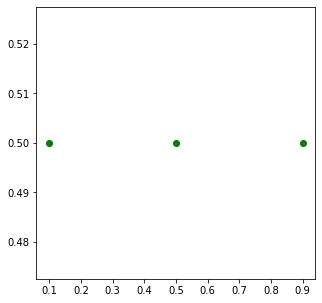Matplotlib provides many specialized functions for different types of plots. scatter() for Scatter Charts, bar() for Bar Charts and so on. You can use them directly, but in practice they are used via higher-level libraries like pandas. In the next section, we will see how to create such charts.

## Exercise

Create a plot that displays the 2 given points with their x,y coordinates with different sumbology.

• point1: Plot it with green color and a triangle marker.
• point2: Plot it with red color and a circle marker.

Reference: matplotlib.pyplot.plot

Hint: You can call plot() multiple times to add new data to the same Axes.

import matplotlib.pyplot as plt

point1 = (4, 1)
point2 = (3, 4)

# Creating Charts## Overview

Pandas allows you to read structured datasets and visualize them using the plot() method. By default, Pandas uses matplotlib to create the plots.

In this notebook, we will take work with open dataset of crime in London.

The following blocks of code will install the required packages and download the datasets to your Colab environment.

import pandas as pd
import os
import matplotlib.pyplot as plt
data_folder = 'data'
output_folder = 'output'

if not os.path.exists(data_folder):
os.mkdir(data_folder)
if not os.path.exists(output_folder):
os.mkdir(output_folder)
def download(url):
filename = os.path.join(data_folder, os.path.basename(url))
if not os.path.exists(filename):
from urllib.request import urlretrieve
local, _ = urlretrieve(url, filename)
print('Downloaded ' + local)

We have 12 different CSV files containing crime data for each month of 2020. We download each of them to the data folder.

files = [
'2020-01-metropolitan-street.csv',
'2020-02-metropolitan-street.csv',
'2020-03-metropolitan-street.csv',
'2020-04-metropolitan-street.csv',
'2020-05-metropolitan-street.csv',
'2020-06-metropolitan-street.csv',
'2020-07-metropolitan-street.csv',
'2020-08-metropolitan-street.csv',
'2020-09-metropolitan-street.csv',
'2020-10-metropolitan-street.csv',
'2020-11-metropolitan-street.csv',
'2020-12-metropolitan-street.csv'
]

data_url = 'https://github.com/spatialthoughts/python-dataviz-web/raw/main/data/crime/'

for f in files:
url = os.path.join(data_url + f)
download(url)

## Data Pre-Processing

It will be helpful to merge all 12 CSV files into a single dataframe. We can use pd.concat() to merge a list of dataframes.

dataframe_list = []

for f in files:
filepath = os.path.join(data_folder, f)
dataframe_list.append(df)

merged_df = pd.concat(dataframe_list)

The resulting dataframe consists of over 1 million records of various crimes recorded in London in the year 2020.

merged_df

## Create a Pie-Chart

Let’s create a pie-chart showing the distribution of different types of crime. Pandas groupby() function allows us to calculate group statistics.

type_counts = merged_df.groupby('Crime type').size()
type_counts

We now uses the plot() method to create the chart. This method is a wrapper around matplotlib and can accept supported arguments from it.

Reference: pandas.DataFrame.plot

fig, ax = plt.subplots(1, 1)
fig.set_size_inches(15,10)
type_counts.plot(kind='pie', ax=ax)
plt.show()

Let’s customize the chart. First we use set_title() method to add a title to the chart and set_ylabel() to remove the empty y-axis label. Lastly, we use the plt.tight_layout() to remove the extra whitespace around the plot.

fig, ax = plt.subplots(1, 1)
fig.set_size_inches(15,10)

type_counts.plot(kind='pie', ax=ax)

ax.set_title('Crime Types', fontsize = 18)
ax.set_ylabel('')

plt.tight_layout()
plt.show()

Matplotlib plots offer unlimited possibilities to customize your charts. Let’s see some of the options available to customize the pie-chart.

• wedgeprops: Customize the look of each ‘wedge’ of the pie.
• textprops: Set the text properties of labels.

Reference: matplotlib.pyplot.pie

wedgeprops={'linewidth': 1, 'edgecolor': 'white'}
textprops= {'fontsize': 10, 'fontstyle': 'italic'}

fig, ax = plt.subplots(1, 1)
fig.set_size_inches(15,10)

type_counts.plot(kind='pie', ax=ax,
wedgeprops=wedgeprops,
textprops=textprops
)

ax.set_title('Crime Types', fontsize = 18)
ax.set_ylabel('')

plt.tight_layout()
plt.show()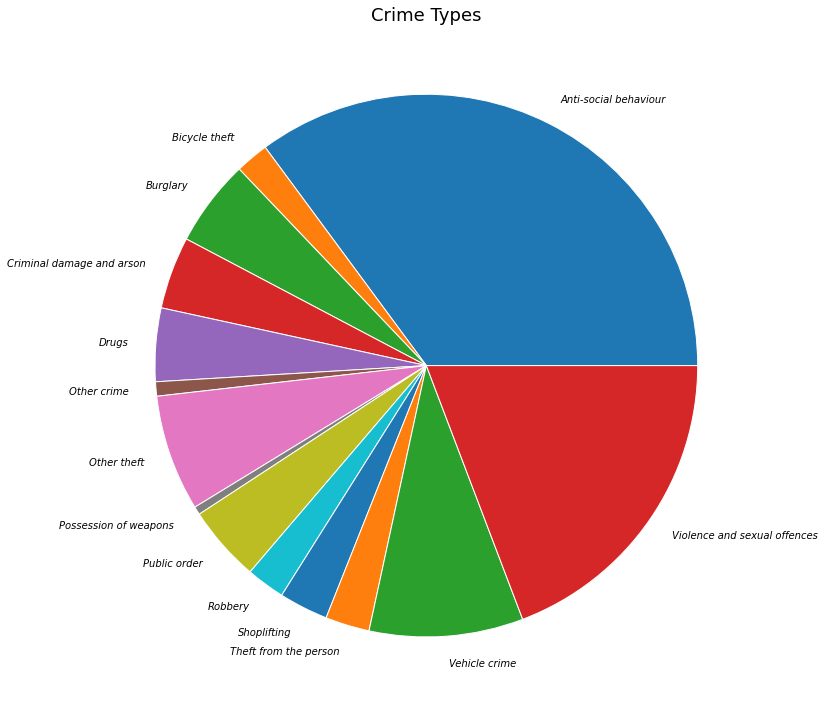## Create a Bar Chart

We can also chart the trend of crime over the year. For this, let’s group the data by month.

monthly_counts = merged_df.groupby('Month').size()
monthly_counts
fig, ax = plt.subplots(1, 1)
fig.set_size_inches(15,7)
monthly_counts.plot(kind='bar', ax=ax)
plt.show()

As we learnt earlier, we can add multiple plots on the same Axes. We can add a line chart along with the bar chart to show the trendline as well. Lastly we add the titles and axis labels to finish the chart.

fig, ax = plt.subplots(1, 1)
fig.set_size_inches(15,7)

monthly_counts.plot(kind='bar', ax=ax)
monthly_counts.plot(kind='line', ax=ax, color='red', marker='o')

ax.set_title('Total Crime by Month')
ax.set_ylabel('Total Incidents')

plt.show()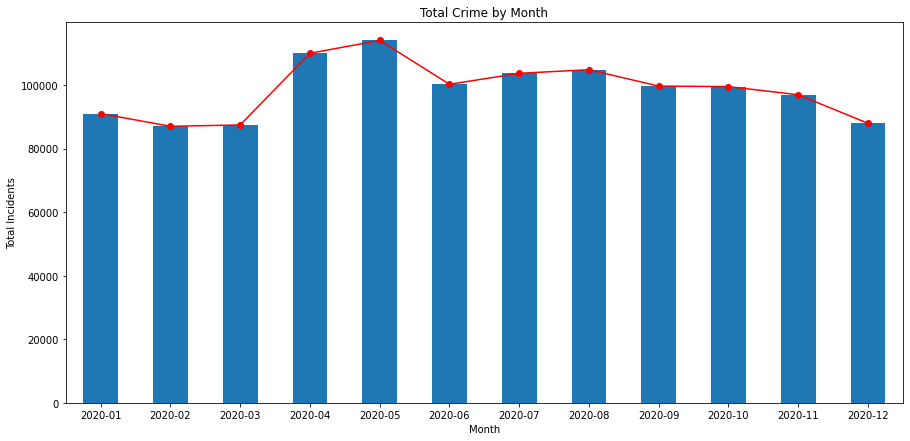## Exercise

Plot the trend of Bicycle thefts as a line chart. The cell below filters the merged_df dataframe to select incidents of ‘Bicycle theft’. Group the results by months and plot the results.

bicycle_thefts = merged_df[merged_df['Crime type'] == 'Bicycle theft']

# Creating Maps## Overview

Similar to Pandas, GeoPandas has a plot() method that can plot geospatial data using Matplotlib.

We will work with census data to create a choropleth map of population density. We will start with a shapefile of census tracts, and join it with tabular data to get a GeoDataframe with census tract geometry and correponding populations.

The following blocks of code will install the required packages and download the datasets to your Colab environment.

%%capture
!apt install libspatialindex-dev
!pip install fiona shapely pyproj rtree mapclassify
!pip install geopandas
import geopandas as gpd
import matplotlib.pyplot as plt
import os
import pandas as pd
data_folder = 'data'
output_folder = 'output'

if not os.path.exists(data_folder):
os.mkdir(data_folder)
if not os.path.exists(output_folder):
os.mkdir(output_folder)
def download(url):
filename = os.path.join(data_folder, os.path.basename(url))
if not os.path.exists(filename):
from urllib.request import urlretrieve
local, _ = urlretrieve(url, filename)
print('Downloaded ' + local)

We will download the the census tracts shapefile and a CSV file containing a variety of population statistics for each tract.

shapefile_name = 'tl_2019_06_tract'
shapefile_exts = ['.shp', '.shx', '.dbf', '.prj']
data_url = 'https://github.com/spatialthoughts/python-dataviz-web/raw/main/data/census/'

for ext in shapefile_exts:
url = data_url + shapefile_name + ext

csv_name = 'ACSST5Y2019.S0101_data.csv'
download(data_url + csv_name)

## Data Pre-Processing

Let’s read the census tracts shapefile and the CSV file containing population counts.

shapefile_path = os.path.join(data_folder, shapefile_name + '.shp')
tracts

We now read the file containing a variety of population statistics for each tract. We read this file as a Pandas DataFrame. The CSV file contains an extra row before the header, so we specify skiprows= to skip reading it.

csv_path = os.path.join(data_folder, csv_name)
table

To join this DataFrame with the GeoDataFrame, we need a column with unique identifiers. We use the GEOID column and process the id so they match exactly in both datasets.

filtered = table[['GEO_ID','NAME', 'S0101_C01_001E']]
filtered = filtered.rename(columns = {'S0101_C01_001E': 'Population', 'GEO_ID': 'GEOID'})

filtered['GEOID'] = filtered.GEOID.str[-11:]

Finally, we do a table join using the merge method.

gdf = tracts.merge(filtered, on='GEOID')
gdf

For creating a choropleth map, we must normalize the population counts. US Census Bureau recommends calculating the population density by dividing the total population by the land area. The original shapefile contains a column ALAND with the land area in square kilometers. Using it, we compute a new column density containing the persons per square kilometer.

gdf['density'] = 1e6*gdf['Population']/gdf['ALAND']
gdf

## Create a Choropleth Map

The plot() method will render the data to a plot.

Reference: geopandas.GeoDataFrame.plot

fig, ax = plt.subplots(1, 1)
fig.set_size_inches(10,10)
gdf.plot(ax=ax)
plt.show()

You can supply additional style options to change the appearance of the map. facecolor and edgecolor parameters are used to determine the fill and outline colors respectively. The stroke width can be adjusted using the linewidth parameter.

fig, ax = plt.subplots(1, 1)
fig.set_size_inches(10,10)
gdf.plot(ax=ax, facecolor='#f0f0f0', edgecolor='#de2d26', linewidth=0.5)

plt.show()

We have the population density for each tract in the density column. We can assign a color to each polygon based on the value in this column - resulting in a choropleth map. Additionally, we need to specify a color ramp using cmap and classification scheme using scheme. The classification schedule will determine how the continuous data will be classified into discrete bins.

Tip: You can add _r to any color ramp name to get a reversed version of that ramp.

References: - Matplotlib Colormaps - Mapclassify Classification Schemes

fig, ax = plt.subplots(1, 1)
fig.set_size_inches(10,10)
gdf.plot(ax=ax, column='density', cmap='RdYlGn_r', scheme='quantiles')
plt.show()

Instead of the class breaks being determined by the classification scheme, we can also manually specify the ranges. This is preferable so we can have a human-interpretable legend. The legend=True parameter adds a legend to our plot.

fig, ax = plt.subplots(1, 1)
fig.set_size_inches(10,10)
gdf.plot(ax=ax, column='density', cmap='RdYlGn_r', scheme='User_Defined',
classification_kwds=dict(bins=[1,10,25,50,100, 250, 500, 1000, 5000]),
legend=True)

plt.show()

We give final touches to our map and save the result as a PNG file. Remember to call plt.savefig() before showing the plot as the plot gets emptied after being shown.

output_path = os.path.join(output_folder, 'california_pop.png')

fig, ax = plt.subplots(1, 1)
fig.set_size_inches(10,10)
gdf.plot(ax=ax, column='density', cmap='RdYlGn_r', scheme='User_Defined',
classification_kwds=dict(bins=[1,10,25,50,100, 250, 500, 1000, 5000]),
legend=True)
ax.set_axis_off()
ax.set_title('California Population Density (2019)', size = 18)

plt.savefig(output_path, dpi=300)

plt.show()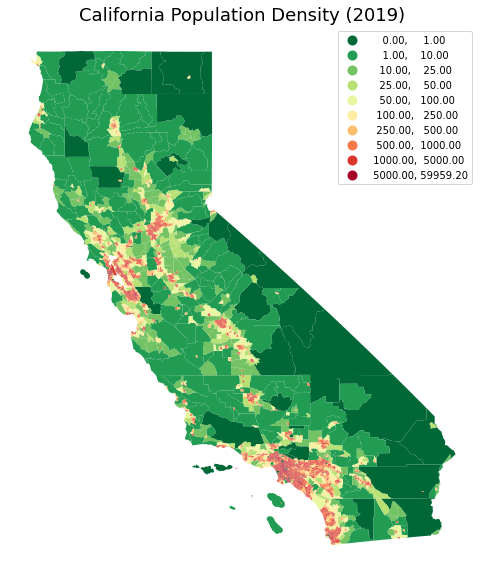## Exercise

Plot the census tracts geodataframe tracts with just outlines and no fill color.

Hint: Set the facecolor option to 'none' for no fills. Check the style_kwds parameter of the plot() method for more details.

fig, ax = plt.subplots(1, 1)
fig.set_size_inches(10,10)
tracts.plot(ax=ax)
plt.show()

# Using Basemaps## Overview

Creating geospatial visualizations oftern require overlaying your data on a basemap. Contextily is a package that allows you to fetch various basemaps from the internet and ad them to your plot as static images.

We will learn how to take a shapefile showing the path of the 2017 Solar Eclipse and create a map with a topographic basemap.

The following blocks of code will install the required packages and download the datasets to your Colab environment.

%%capture
!apt install libspatialindex-dev
!pip install fiona shapely pyproj rtree
!pip install geopandas
!pip install contextily
import contextily as cx
import geopandas as gpd
import os
import matplotlib.pyplot as plt
data_folder = 'data'
output_folder = 'output'

if not os.path.exists(data_folder):
os.mkdir(data_folder)
if not os.path.exists(output_folder):
os.mkdir(output_folder)
def download(url):
filename = os.path.join(data_folder, os.path.basename(url))
if not os.path.exists(filename):
from urllib.request import urlretrieve
local, _ = urlretrieve(url, filename)

path_shapefile = 'upath17'
umbra_shapefile = 'umbra17'
shapefile_exts = ['.shp', '.shx', '.dbf', '.prj']
data_url = 'https://github.com/spatialthoughts/python-dataviz-web/raw/main/data/eclipse/'

for shapefile in [path_shapefile, umbra_shapefile]:
for ext in shapefile_exts:
url = data_url + shapefile + ext
download(url)

## Data Pre-Processing

path_shapefile_path = os.path.join(data_folder, path_shapefile + '.shp')
path_gdf
umbra_shapefile_path = os.path.join(data_folder, umbra_shapefile + '.shp')
umbra_gdf[:5]

## Create a Multi-Layer Map

We can show a GeoDataFrame using the plot() method.

fig, ax = plt.subplots(1, 1)
fig.set_size_inches(15,7)
path_gdf.plot(ax=ax, facecolor='#cccccc', edgecolor='#969696', alpha=0.5)
plt.show()

To add another layer to our plot, we can simply render another GeoDataFrame on the same Axes.

fig, ax = plt.subplots(1, 1)
fig.set_size_inches(15,7)
path_gdf.plot(ax=ax, facecolor='#cccccc', edgecolor='#969696', alpha=0.5)
umbra_gdf.plot(ax=ax, facecolor='#636363', edgecolor='none')
plt.show()

The visualization is not useful as it is missing context. We want to overlay this on a basemap to understand where the eclipse was visible from. We can choose from a variety of basemap tiles. There are over 200 basemap styles included in the library. Let’s see them using the providers property.

providers = cx.providers
providers

For overlaying the eclipse path, let’s use the OpenTopoMap basemap. We need to specify a CRS for the map. For now, let’s use the CRS of the original shapefile.

fig, ax = plt.subplots(1, 1)
fig.set_size_inches(15,7)

path_gdf.plot(ax=ax, facecolor='#cccccc', edgecolor='#969696', alpha=0.5)
umbra_gdf.plot(ax=ax, facecolor='#636363', edgecolor='none')

plt.show()

The web tiles for the basemap are in the Web Mercator CRS (EPSG:3857). When you request them in a different CRS, they are warped to the requested CRS. This may cause the labels to not be legible in some cases. Instead, we can request the tiles in their original CRS and reproject our data layer to its CRS.

path_reprojected = path_gdf.to_crs('EPSG:3857')
umbra_reprojected = umbra_gdf.to_crs('EPSG:3857')

fig, ax = plt.subplots(1, 1)
fig.set_size_inches(15,7)

path_reprojected.plot(ax=ax, facecolor='#cccccc', edgecolor='#969696', alpha=0.5)
umbra_reprojected.plot(ax=ax, facecolor='#636363', edgecolor='none')

ax.set_axis_off()
ax.set_title('2017 Total Solar Eclipse Path', size = 18)

plt.show()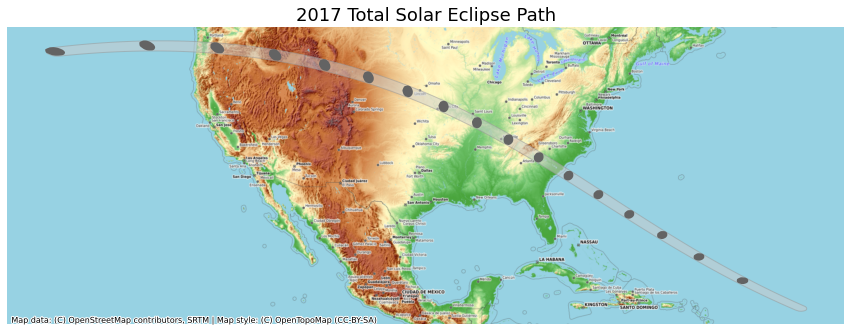## Exercise

Instead of the OpenTopoMap, create a visualization using the Toner basemap from Stamen. Save the resulting visualization as a PNG file eclipse_path.png.

# XArray Basics## Overview

Many climate and meteorological datasets come as gridded rasters in data formats such as NetCDF and GRIB. We will use XArray to read, process and visualize the gridded raster dataset.

Xarray is an evolution of rasterio and is inspired by libraries like pandas to work with raster datasets. It is particularly suited for working with multi-dimensional time-series raster datasets. It also integrates tightly with dask that allows one to scale raster data processing using parallel computing. XArray provides Plotting Functions based on Matplotlib.

In this section, we will take the Gridded Monthly Temperature Anomaly Data from 1880-present from GISTEMP and visualize the temperature anomaly for the year 2021.

The following blocks of code will install the required packages and download the datasets to your Colab environment.

import os
import matplotlib.pyplot as plt
import xarray as xr
data_folder = 'data'
output_folder = 'output'

if not os.path.exists(data_folder):
os.mkdir(data_folder)
if not os.path.exists(output_folder):
os.mkdir(output_folder)
def download(url):
filename = os.path.join(data_folder, os.path.basename(url))
if not os.path.exists(filename):
from urllib.request import urlretrieve
local, _ = urlretrieve(url, filename)

data_url = 'https://github.com/spatialthoughts/python-dataviz-web/raw/main/data/gistemp/'

download(data_url + filename)

## XArray Terminology

By convention, XArray is imported as xr. We use Xarray’s open_dataset() method to read the gridded raster. The result is a xarray.Dataset object.

file_path = os.path.join(data_folder, filename)
ds = xr.open_dataset(file_path)
ds

The NetCDF file contains a grid of values for each month from 1880-2021 at a spatial resolution of 2 degrees. Let’s understand what is contained in a Dataset.

• Variables: This is similar to a band in a raster dataset. Each variable contains an array of values.
• Dimensions: This is similar to number of array axes.
• Coordinates: These are the labels for values in each dimension.
• Attributes: This is the metadata associated with the dataset.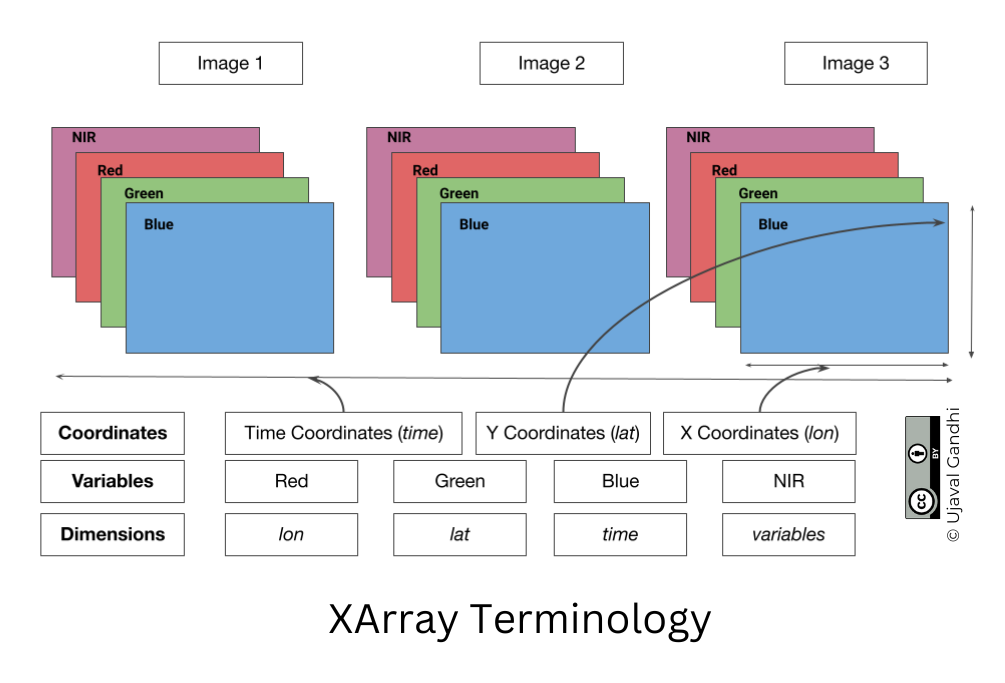A Dataset consists of one or more xarray.DataArray object. This is the main object that consists of a single variable with dimension names, coordinates and attributes. You can access each variable using dataset.variable_name syntax.

Let’s see the time_bnds variable. This contains a 2d array which has both a starting and ending time for each one averaging period.

ds.time_bnds

The main variable of interest is = tempanomaly - containing the grid of temperature anomaly values at different times. Let’s select that variable and store it as da.

da = ds.tempanomaly
da

## Selecting Data

XArray provides a very powerful way to select subsets of data, using similar framework as Pandas. Similar to Panda’s loc and iloc methods, XArray provides sel and isel methods. Since DataArray dimensions have names, these methods allow you to specify which dimension to query.

Let’s select the temperature anomany values for the last time step. Since we know the index (-1) of the datam we can use isel method.

da.isel(time=-1)

We can also specify a value to query using the sel() method.

da.sel(time='2021-12-15')

We can specify multiple dimensions to query for a subset. Let’s extract the temperature anomaly at lat=49, lon=-123 and time='2021-06-15'. This region experienced abnormally high temperatures in June 2021.

da.sel(lat=49, lon=-123, time='2021-06-15')

The sel() method also support nearest neighbor lookups. This is useful when you do not know the exact label of the dimension, but want to find the closest one.

Tip: You can use interp() instead of sel() to interpolate the value instead of closest lookup.

da.sel(lat=28.6, lon=77.2, time='2021-05-01', method='nearest')

You can call .values on a DataArray to get an array of the values.

selected = da.sel(lat=28.6, lon=77.2, time='2021-05-01', method='nearest')
print(selected.values)

The sel() method also allows specifying range of values using Python’s built-in slice() function. The code below will select all observationss in the year 2021.

da.sel(time=slice('2021-01-01', '2021-12-31'))

XArray has a where() function that allows you to extract a subset of the array. The code block below extracts the anomaly at a specific Lat/Lon. We then use the .where() function to select items that have a positive anomaly.

selected = da.sel(lat=28.6, lon=77.2, method='nearest')
selected

We can use drop=True to remove all items where the condition did not match and create a subset of the data.

positive = selected.where(selected > 0, drop=True)
positive

## Aggregating Data

A very-powerful feature of XArray is the ability to easily aggregate data across dimensions - making it ideal for many remote sensing analysis. Let’s calculate the average temperature anomany for the year 2021.

We first select the subset for year 2021 and apply the .mean() aggregation across the time dimension.

subset2021 = da.sel(time=slice('2021-01-01', '2021-12-31'))
subset2021.mean(dim='time')

XArray has many features easily work with time-series data such as this. We can use temporal components to aggregate the data across time. Here we take our monthly time-series of anomalies and aggregate it to a yearly time-series using the groupby() method.

Reference: Resampling and grouped operations

yearly = da.groupby('time.year').mean(dim='time')
yearly

## Exercise

Can you find out when did the highest temperature anomaly occured at a specific location?

Replace the lat and lon in the following code with your chosen location. You will see the resulting max_anomaly DataArray with the anomaly value along with lat, lon and time coordinates. Extract the time coordinate of the resulting array and print the time when the maximum anomaly occured.

selected = da.sel(lat=28.6, lon=77.2, method='nearest')
max_anomaly = selected.where(selected==selected.max(), drop=True)
max_anomaly

# Mapping Gridded Datasets## Overview

In this section, we will take the Gridded Monthly Temperature Anomaly Data from 1880-present from GISTEMP and visualize the temperature anomaly for the year 2021.

The following blocks of code will install the required packages and download the datasets to your Colab environment.

%%capture
!apt-get -qq remove python-shapely python3-shapely
!pip install --no-binary shapely shapely --force
!pip install --no-binary cartopy cartopy==0.19.0.post1
import cartopy
import cartopy.crs as ccrs
import os
import matplotlib.pyplot as plt
import xarray as xr
data_folder = 'data'
output_folder = 'output'

if not os.path.exists(data_folder):
os.mkdir(data_folder)
if not os.path.exists(output_folder):
os.mkdir(output_folder)
def download(url):
filename = os.path.join(data_folder, os.path.basename(url))
if not os.path.exists(filename):
from urllib.request import urlretrieve
local, _ = urlretrieve(url, filename)

data_url = 'https://github.com/spatialthoughts/python-dataviz-web/raw/main/data/gistemp/'

download(data_url + filename)

## Data Pre-Processing

We read the data using XArray, select the tempanomaly variable and aggregate it to yearly anoamalies.

file_path = os.path.join(data_folder, filename)
ds = xr.open_dataset(file_path)
da = ds.tempanomaly
yearly = da.groupby('time.year').mean(dim='time')
yearly

## Plotting using Matplotlib

XArray provides a plot() method based on Matplotlib. When you call plot() on a 2D DataArray, it uses the xarray.plot.pcolormesh() function that creates a Pseudocolor plot.

Reference: xarray.plot

anomaly2021 = yearly.sel(year=2021)
anomaly2021
fig, ax = plt.subplots(1, 1)
fig.set_size_inches(15, 7)
anomaly2021.plot(ax=ax)
plt.show()

While the plot() method works, it is slower than the plot.imshow() method when rendering large 2D-arrays. So we would prefer using it instead of the plot() method.

Reference : xarray.plot.imshow

fig, ax = plt.subplots(1, 1)
fig.set_size_inches(15, 7)
anomaly2021.plot.imshow(ax=ax)
plt.show()

We can customize the plot using Matplotlib’s options.

fig, ax = plt.subplots(1, 1)
fig.set_size_inches(15, 7)

anomaly2021.plot.imshow(ax=ax,

ax.set_title('Temperature Anomaly in 2021 (°C)', fontsize = 14)

plt.show()

## Plotting using CartoPy

To create more informative map visualization, we need to reproject this grid to another projection. CartoPy supports a wide range of projections and can plot them using matplotlib. CartoPy creates a GeoAxes object and replaces the default Axes with it. This allows you to plot the data on a specified projection.

We start as usual by create a subplot but specify an additional argument to set the CRS from CartoPy.

Reference: CartoPy List of Projections

fig, ax = plt.subplots(1, 1, subplot_kw={'projection': ccrs.Orthographic(0, 30)})
fig.set_size_inches(5,5)

anomaly2021.plot.imshow(ax=ax,
vmin=-3, vmax=3, cmap='coolwarm',
transform=ccrs.PlateCarree())

plt.tight_layout()
plt.show()

We can further customize the map by adjusting the colorbar.

Reference: matplotlib.pyplot.colorbar

cbar_kwargs = {
'orientation':'horizontal',
'fraction': 0.025,
'extend':'neither'
}

fig, ax = plt.subplots(1, 1, subplot_kw={'projection': ccrs.Orthographic(0, 30)})
fig.set_size_inches(10, 10)
anomaly2021.plot.imshow(
ax=ax,
vmin=-3, vmax=3, cmap='coolwarm',
transform=ccrs.PlateCarree(),
cbar_kwargs=cbar_kwargs)

ax.coastlines()
plt.title('Temperature Anomaly in 2021 (°C)', fontsize = 14)

output_folder = 'output'
output_path = os.path.join(output_folder, 'anomaly.jpg')
plt.savefig(output_path, dpi=300)
plt.show()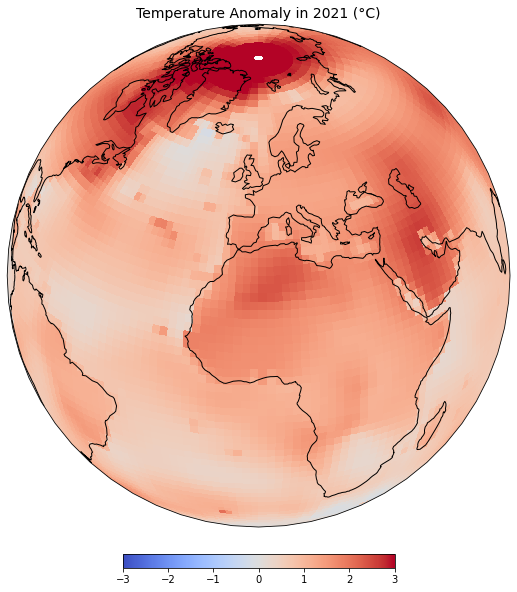## Exercise

Display the map in the Robinson projection.

# Visualizing Rasters## Overview

In the previous notebook, we learnt how to use Xarray to work with gridded datasets. XArray is also well suited to work with georeferenced rasters - such as satellite imagery, population grids, or elevation data.rioxarray is an extension of xarray that makes it easy to work with geospatial rasters. You can install the rioxarray package from the conda-forge channel.

In this section, we will take 4 individual SRTM tiles around the Mt. Everest region and merge them to a single GeoTiff using RasterIO. We will also use matplotlib to visualize the result with some annonations.

The following blocks of code will install the required packages and download the datasets to your Colab environment.

%%capture
!pip install --quiet rioxarray

By convention, rioxarray is imported as rxr.

Remember to always import rioxarray even if you are using sub-modules such as merge_arrays. Importing rioxarray activates the rio accessor which is required for all operations.

import os
import rioxarray as rxr
from rioxarray.merge import merge_arrays
import matplotlib.pyplot as plt
data_folder = 'data'
output_folder = 'output'

if not os.path.exists(data_folder):
os.mkdir(data_folder)
if not os.path.exists(output_folder):
os.mkdir(output_folder)
def download(url):
filename = os.path.join(data_folder, os.path.basename(url))
if not os.path.exists(filename):
from urllib.request import urlretrieve
local, _ = urlretrieve(url, filename)

srtm_tiles = [
'N27E086.hgt',
'N27E087.hgt',
'N28E086.hgt',
'N28E087.hgt'
]

data_url = 'https://github.com/spatialthoughts/python-dataviz-web/raw/main/data/srtm/'

for tile in srtm_tiles:
url = '{}/{}'.format(data_url, tile)
download(url)

## Rioxarray Basics

The open_rasterio() method from rioxarray is able to read any data source supported by. the rasterio library. Let’s open a single SRTM tile using rioxarray.

filename = 'N28E087.hgt'
file_path = os.path.join(data_folder, filename)
rds = rxr.open_rasterio(file_path)

The result is a xarray.DataArray object.

rds

You can access the pixel values using the values property which returns the array’s data as a numpy array.

rds.values

A xarray.DataArray object also contains 1 or more coordinates. Each coordinate is a 1-dimensional array representing values along one of the data axes. In case of the 1-band SRTM elevation data, we have 3 coordinates - x, y and band.

rds.coords

The raster metadata is stored in the rio accessor. This is enabled by the rioxarray library which provides geospatial functions on top of xarray.

print('CRS:', rds.rio.crs)
print('Resolution:', rds.rio.resolution())
print('Bounds:', rds.rio.bounds())
print('Width:', rds.rio.width)
print('Height:', rds.rio.height)
band1 = rds.sel(band=1)
band1

## Plotting Multiple Rasters

Open each source file using open_rasterio() method and store the resulting datasets in a list.

datasets = []
for tile in srtm_tiles:
path = os.path.join(data_folder, tile)
rds = rxr.open_rasterio(path)
band = rds.sel(band=1)
datasets.append(band)

You can visualize any DataArray object by calling plot() method. Here we create a subplot with 1 row and 4 columns. The subplots() method will return a list of Axes that we can use to render each of the source SRTM rasters. For plots with multiple columns, the Axes will be a nested list. To easily iterate over it, we can use .flat which returns a 1D iterator on the axes.

While plotting the data, we can use the cmap option to specify a color ramp. Here we are using the built-in Greys ramp. Appending **_r** gives us the inverted ramp with blacks representing lower elevation values.

fig, axes = plt.subplots(1, 4)
fig.set_size_inches(15,3)
for index, ax in enumerate(axes.flat):
da = datasets[index]
im = da.plot.imshow(ax=ax, cmap='Greys_r')
filename = srtm_tiles[index]
ax.set_title(filename)

plt.tight_layout()
plt.show()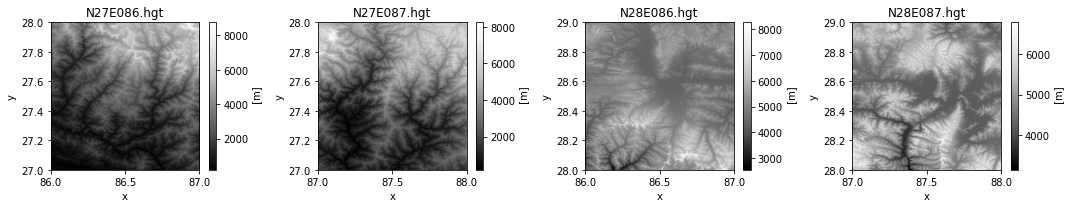## Merging Rasters

Now that you understand the basic data structure of xarray and the &rio* extension, let’s use it to process some data. We will take 4 individual SRTM tiles and merge them to a single GeoTiff. You will note that rioxarray handles the CRS and transform much better - taking care of internal details and providing a simple API.

We will use the merge_arrays() method from the rioxarray.merge module to merge the rasters. We can also specify an optional method that controls how overlapping tiles are merged. Here we have chosen first which takes the value of the first raster in the overlapping region.

Reference: merge_arrays()

merged = merge_arrays(datasets, method='first')
merged

We can now visualize the merged raster.

fig, ax = plt.subplots()
fig.set_size_inches(12, 10)
merged.plot.imshow(ax=ax, cmap='Greys_r')
ax.set_title('merged')
plt.show()

## Annotating Plots

Sometime it is helpful to add annotations on your plot to highlight a feature or add a text label. In this section we will learn how to use the annotate the DEM to show the location and elevation of Mt. Everest.

First, we locate the coordinates of the maximum elevation in the merged DataArray using the max() function. We can then use where() function to filter the elements where the value equals the maximum elevation. Lastly, we run squeeze() to remove the extra empty dimension from the result.

References:

max_da = merged.where(merged==merged.max(), drop=True).squeeze()
max_da

We now extract the x,y coordinates and the value of the maximum elevation.

max_x = max_da.x.values
max_y = max_da.y.values
max_elev = int(max_da.values)
print(max_x, max_y, max_elev)

Now we plot the merged raster and annotate it using the annotate() function.

Reference: matplotlib.pyplot.annotate

fig, ax = plt.subplots(1, 1)
fig.set_size_inches(12, 10)
ax.plot(max_x, max_y, '^r', markersize=11)
ax.annotate('Mt. Everest (elevation:{}m)'.format(max_elev),
xy=(max_x, max_y), xycoords='data',
xytext=(20, 20), textcoords='offset points',
arrowprops=dict(arrowstyle='->', color='black')
)
plt.tight_layout()
plt.show()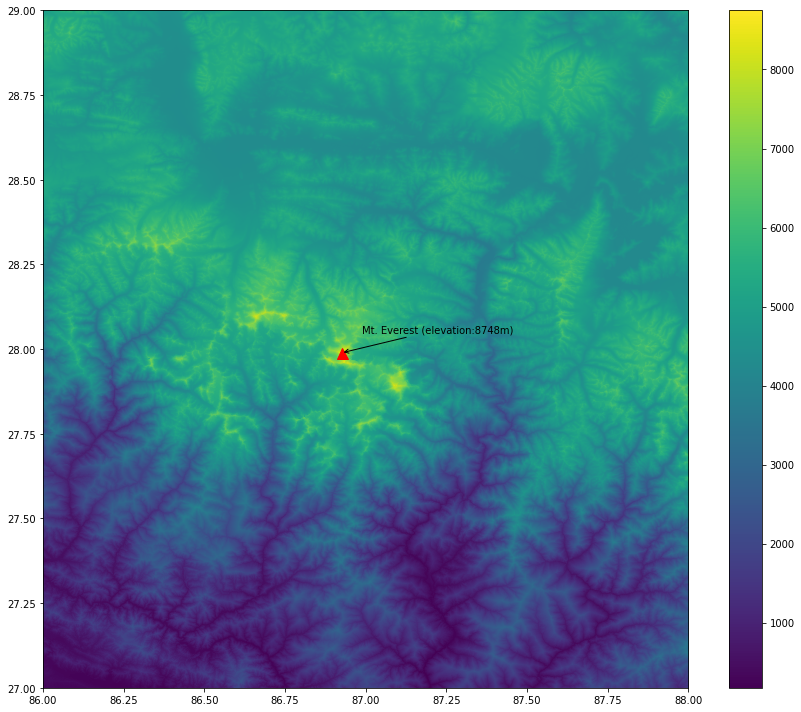Finally, save the merged array to disk as a GeoTiff file.

output_filename = 'merged.tif'
output_path = os.path.join(output_folder, output_filename)
merged.rio.to_raster(output_path)

## Exercise

Add contours to the elevation plot below. You can use the xarray.plot.contour function to create the contour plot.

Hint: Use the options colors=black and levels=10.

fig, ax = plt.subplots(1, 1)
fig.set_size_inches(12, 10)
plt.tight_layout()
plt.show()

# Interactive Maps with Folium## Overview

Folium is a Python library that allows you to create interactive maps based on the popular Leaflet javascript library.

In this section, we will learn how to create an interactive map showing driving directions between two locations.

## Setup

import os
import folium
data_folder = 'data'
output_folder = 'output'

if not os.path.exists(data_folder):
os.mkdir(data_folder)
if not os.path.exists(output_folder):
os.mkdir(output_folder)

We will be using OpenRouteService API to calculate the directions. Please sign-up for a free account and create an API key. If you already have an account, the API key is obtained from the OpenRouteService Dashboard. Enter your API key below.

ORS_API_KEY = ''

## Folium Basics

We will learn the basics of folium by creating an interactive map showing the driving directions between two chosen locations. Let’s start by defining the coordinates of two cities.

san_francisco = (37.7749, -122.4194)
new_york = (40.661, -73.944)

To create a map, we initialize the folium.Map() class which creates a map object with the default basemap. To display the map a Jupyter notebook, we can simply enter the name of the map object.

m = folium.Map()
m

The default map spans the full width of the Jupyter notebook - making it difficult to navigate. The Map() constructor supports width and height parameters that control the size of the leaflet map, but you still end up with a lot of extra empty space below the map. The preferred way to get a map of exact size is to create a Figure first and add the map object to it.

from folium import Figure
fig = Figure(width=800, height=400)
m = folium.Map(location=[39.83, -98.58], zoom_start=4)
fig.add_child(m)

The map object m can be manipulated by adding different elements to it. Contrary to how Matplotlib objects work, the map object does not get emptied when displayed. So you are able to visualize and incrementally add elements to it. Let’s add some markers to the map using folium.map.Marker class.

folium.Marker(san_francisco, popup='San Francisco').add_to(m)

m

The markers can be customized to have a different color or icons. You can check the folium.map.Icon class for options for creating icons. This class supports a vast range of icons from the fontawesome icons and bootstrap icons libraries. You can choose the name of the icon from there to use it in your Folium map. The prefix parameter can be fa for FontAwesome icons or glyphicon for Bootstrap3.

from folium import Figure
fig = Figure(width=800, height=400)
m = folium.Map(location=[39.83, -98.58], zoom_start=4)
folium.Marker(san_francisco, popup='San Francisco',
icon=folium.Icon(
color='green', icon='crosshairs', prefix='fa')
folium.Marker(new_york, popup='New York',
icon=folium.Icon(color='red', icon='crosshairs', prefix='fa')
fig.add_child(m)
import requests

san_francisco = (37.7749, -122.4194)
new_york = (40.661, -73.944)

parameters = {
'api_key': ORS_API_KEY,
'start' : '{},{}'.format(san_francisco, san_francisco),
'end' : '{},{}'.format(new_york, new_york)
}

response = requests.get(
'https://api.openrouteservice.org/v2/directions/driving-car', params=parameters)

if response.status_code == 200:
print('Request successful.')
data = response.json()
else:
print('Request failed.')

Extract the coordinates for the driving directions.

route= data['features']['geometry']['coordinates']
route[:5]

The coordinates returned by OpenRouteService API is in the order [X,Y] (i.e. [Longitude, Latitude]) whereas Folium requires the coordinates in [Y,X] (i.e. [Latitude, Longitude]) order. We can swap them before plotting.

route_xy = []
for x, y in route:
route_xy.append((y,x))
route_xy[:5]

An easier way to accomplish the same is by using a Python List Comprehension.

route_xy = [(y, x) for x, y in route]
route_xy[:5]

We extract the route summary returned by the API which contains the total driving distance in meters.

summary = data['features']['properties']['summary']
distance = round(summary['distance']/1000)
tooltip = 'Driving Distance: {}km'.format(distance)

We can use the folium.vector_layers.Polyline class to add a line to the map. The class has a smooth_factor parameter which can be used to simplify the line displayed when zoomed-out. Setting a higher number results in better performance.

folium.PolyLine(route_xy, tooltip=tooltip, smooth_factor=1).add_to(m)
m

Folium maps can be saved to a HTML file by calling save() on the map object.

output_folder = 'output'
if not os.path.exists(output_folder):
os.mkdir(output_folder)
output_path = os.path.join(output_folder, 'directions.html')
m.save(output_path)

## Exercise

Below is the complete code to create an interactive map with the driving directions between two cities. Replace the origin and destination with your chosen cities and create an interactive map.

import folium
import requests

###############################
###  Replace Variables Below
###############################
origin = (37.7749, -122.4194)
origin_name = 'San Francisco'
destination = (40.661, -73.944)
destination_name = 'New York'
map_center = (39.83, -98.58)
ORS_API_KEY = ''
###############################
parameters = {
'api_key': ORS_API_KEY,
'start' : '{},{}'.format(origin, origin),
'end' : '{},{}'.format(destination, destination)
}

response = requests.get(
'https://api.openrouteservice.org/v2/directions/driving-car', params=parameters)

if response.status_code == 200:
print('Request successful.')
data = response.json()
else:
print('Request failed.')

route= data['features']['geometry']['coordinates']
summary = data['features']['properties']['summary']

route_xy = [(y, x) for x, y in route]
distance = round(summary['distance']/1000)
tooltip = 'Driving Distance: {}km'.format(distance)

from folium import Figure
fig = Figure(width=800, height=400)

m = folium.Map(location=map_center, zoom_start=4)
folium.Marker(origin, popup=origin_name,
icon=folium.Icon(
color='green', icon='crosshairs', prefix='fa')
folium.Marker(destination, popup=destination_name,
icon=folium.Icon(color='red', icon='crosshairs', prefix='fa')
fig.add_child(m)

# Multi-layer Interactive MapsOpen the notebook named 09_multilayer_maps.ipynb.

## Overview

Folium supports creating maps with multiple layers. Recent versions of GeoPandas have built-in support to create interactive folium maps from a GeoDataFrame.

In this section, we will create a multi-layer interactive map using 2 vector datasets.

%%capture
!apt install libspatialindex-dev
!pip install fiona shapely pyproj rtree mapclassify
!pip install geopandas
import os
import folium
from folium import Figure
import geopandas as gpd
data_folder = 'data'
output_folder = 'output'

if not os.path.exists(data_folder):
os.mkdir(data_folder)
if not os.path.exists(output_folder):
os.mkdir(output_folder)
def download(url):
filename = os.path.join(data_folder, os.path.basename(url))
if not os.path.exists(filename):
from urllib.request import urlretrieve
local, _ = urlretrieve(url, filename)

filename = 'karnataka.gpkg'
data_url = 'https://github.com/spatialthoughts/python-dataviz-web/raw/main/data/osm/'
download(data_url + filename)

## Using GeoPandas explore()

data_pkg_path = 'data'
filename = 'karnataka.gpkg'
path = os.path.join(data_pkg_path, filename)
state_gdf = gpd.read_file(path, layer='karnataka')

We can use the explore() method to create an interactive folium map from the GeoDataFrame. When you call explore() a folium object is created. You can save that object and use it to display or add more layers to the map.

m = districts_gdf.explore()
m

The default output of the explore() method is a full-width folium map. If you need more control, a better approach is to create a follium.Figure object and add the map to it. For this approach we need to first compute the extent of the map.

bounds = districts_gdf.total_bounds
bounds

Now we can create a figure of the required size, and add a folium map to it. The explore() function takes a m agrument where we can supply an existing folium map to which to render the GeoDataFrame.

fig = Figure(width=800, height=400)

m = folium.Map()
m.fit_bounds([[bounds,bounds], [bounds,bounds]])

districts_gdf.explore(m=m)

fig.add_child(m)

Folium supports a variety of basemaps. Let’s change the basemap to use Stamen Terrain tiles. Additionally, we can change the styling using the color and style_kwds parameters.

fig = Figure(width=800, height=400)

m = folium.Map(tiles='Stamen Terrain')
m.fit_bounds([[bounds,bounds], [bounds,bounds]])

districts_gdf.explore(
m=m,
color='black',
style_kwds={'fillOpacity': 0.3, 'weight': 0.5},
)

fig.add_child(m)

Let’s add the roads_gdf layer to the map.

roads_gdf

The GeoDataFrame contains roads of different categories as given in the ref column. Let’s add a category column so we can use it to apply different styles to each category of the road.

def get_category(row):
ref = str(row['ref'])
if 'NH' in ref:
return 'NH'
elif 'SH' in ref:
return 'SH'
else:
return 'NA'

roads_gdf
fig = Figure(width=800, height=400)

m = folium.Map()
m.fit_bounds([[bounds,bounds], [bounds,bounds]])

m=m,
column='category',
categories=['NH', 'SH'],
cmap=['#1f78b4', '#e31a1c'],
categorical=True
)

fig.add_child(m)

## Create Multi-layer Maps

When you call explore() a folium object is created. You can save that object and add more layers to the same object.

fig = Figure(width=800, height=400)

m = folium.Map(tiles='Stamen Terrain')
m.fit_bounds([[bounds,bounds], [bounds,bounds]])

districts_gdf.explore(
m=m,
color='black',
style_kwds={'fillOpacity': 0.3, 'weight':0.5},
name='districts',
tooltip=False)

m=m,
column='category',
categories=['NH', 'SH'],
cmap=['#1f78b4', '#e31a1c'],
categorical=True,
name='highways'
)

fig.add_child(m)

To make our map easier to explore, we also add a Layer Control that displays the list of layers on the top-right corner and also allows the users to turn them on or off. The name parameter to the explore() function controls the name that will be displayed in the layer control.

folium.LayerControl().add_to(m)
m

## Exercise

Add the state_gdf layer to the folium map below with a thick blue border and no fill. Save the resulting map as a HTML file on your computer.

Hint: Use the style_kwds with ‘fill’ and ‘weight’ options.

fig = Figure(width=800, height=400)

m = folium.Map(tiles='Stamen Terrain')
m.fit_bounds([[bounds,bounds], [bounds,bounds]])

districts_gdf.explore(
m=m,
color='black',
style_kwds={'fillOpacity': 0.3, 'weight':0.5},
name='districts',
tooltip=False)

m=m,
column='category',
categories=['NH', 'SH'],
cmap=['#1f78b4', '#e31a1c'],
categorical=True,
name='highways'
)

m

# Leafmap Basics## Overview

Leafmap is a Python package for interactive mapping that supports a wide-variety of plotting backends.

We will explore the capabilities of Leafmap and create a map that includes vector and raster layers. For a more comprehensive overview, check out leafmap key Features.

%%capture
!apt install libspatialindex-dev
!pip install fiona shapely pyproj rtree mapclassify
!pip install geopandas
!pip install leafmap
import os
import geopandas as gpd
import leafmap.foliumap as leafmap
data_folder = 'data'
output_folder = 'output'

if not os.path.exists(data_folder):
os.mkdir(data_folder)
if not os.path.exists(output_folder):
os.mkdir(output_folder)
def download(url):
filename = os.path.join(data_folder, os.path.basename(url))
if not os.path.exists(filename):
from urllib.request import urlretrieve
local, _ = urlretrieve(url, filename)

json_file = 'bangalore_wards.json'

data_url = 'https://github.com/spatialthoughts/python-dataviz-web/raw/main/data/bangalore/'

for f in json_file, gpkg_file:
download(data_url + f)

## Creating a Map

m = leafmap.Map(width=800, height=500)
m

You can change the basemap to a Google basemap and set the center of the map. leafmap.Map() supports many arguments to customize the map and available controls.

References:

m = leafmap.Map(width=800, height=500, google_map='HYBRID', center=(12.97, 77.59), zoom=10)
m

Leafmap’s foliummap module supports adding a variety of data types along with helper functions such as set_center(). Let’s add a GeoJSON file to the map using add_geojson().

m = leafmap.Map(width=800, height=500)

json_filepath = os.path.join(data_folder, json_file)

m.set_center(77.59, 12.97, 10)
m

We can also add any vector layer that can be read by GeoPandas. Here we add a line layer from a GeoPackage using the add_gdf() function. The styling parameters can be any folium supported styles.

m = leafmap.Map(width=800, height=500)

gpkg_filepath = os.path.join(data_folder, gpkg_file)

m

A very useful feature of LeafMap is the ability to load a Cloud-Optimized GeoTIFF (COG) file directly from a URL without the need of a server. The file is streamed directly and high-resolution tiles are automatically fetched as you zoom in. We load a 8-bit RGB image hosted on GitHub using the add_cog_layer() function.

Reference: leafmap.Map.add_cog_layer

m = leafmap.Map(width=800, height=500)

cog_url = os.path.join(data_url, 'bangalore_lulc_rgb.tif')
bounds = leafmap.cog_bounds(cog_url)

m.zoom_to_bounds(bounds)
m

Leafmap also supports adding custom legends. We define a list of color codes and labels for the land cover classes contained in the bangalore_lulc.tif image. We can now add a legend to the map using the add_legend() function.

m = leafmap.Map(width=800, height=500)

cog_url = os.path.join(data_url, 'bangalore_lulc_rgb.tif')
bounds = leafmap.cog_bounds(cog_url)

m.zoom_to_bounds(bounds)

colors = ['#006400', '#ffbb22','#ffff4c','#f096ff','#fa0000',
'#b4b4b4','#f0f0f0','#0064c8','#0096a0','#00cf75','#fae6a0']
labels = ["Trees","Shrubland","Grassland","Cropland","Built-up",
"Barren / sparse vegetation","Snow and ice","Open water",
"Herbaceous wetland","Mangroves","Moss and lichen"]
m

We can save the resulting map to a HTML file using the to_html() function.

m = leafmap.Map(width=800, height=500)

cog_url = os.path.join(data_url, 'bangalore_lulc_rgb.tif')
bounds = leafmap.cog_bounds(cog_url)

m.zoom_to_bounds(bounds)

colors = ['#006400', '#ffbb22','#ffff4c','#f096ff','#fa0000',
'#b4b4b4','#f0f0f0','#0064c8','#0096a0','#00cf75','#fae6a0']
labels = ["Trees","Shrubland","Grassland","Cropland","Built-up",
"Barren / sparse vegetation","Snow and ice","Open water",
"Herbaceous wetland","Mangroves","Moss and lichen"]

output_file = 'lulc.html'
output_path = os.path.join(output_folder, output_file)
m.to_html(output_path)

## Exercise

The code below contains a basic leafmap map. We want to a raster layers of VIIRS Nighttime Lights over India. The URL to a Cloud Optmized GeoTiff (COG) file hosted on Google Cloud Storage is given below.

Add the data to the map and visualize it.

Reference: leafmap.Map.add_cog_layer

You will need to specify additional kwargs parameters to create a correct visualization.

1. The GeoTIFF image is a single-band image with grayscale values of night light intensities. The range of these values are between 0-60. Use rescale='0,60'.
2. The image has a nodata values stored as nan. Use nodata='nan'.
3. A grayscale image can be displayed in color using a colormap. Use colormap_name=viridis.
gcs_bucket = 'https://storage.googleapis.com/spatialthoughts-public-data/'
cog_url = os.path.join(gcs_bucket, 'viirs_ntl_2021_india.tif')

# Streamlit Basics

Streamlit is a Python library that allows you to create web-apps and dashboard by just writing Python code. It provides you with a wide range of UI widgets and layouts that can be used to build user- interfaces. Your streamlit app is just a regular Python file with a .py extension.

## Installation and Setting up the Environment

You need to install the streamlit package to create the app. We will be using Anaconda to install streamlit and related packages on your machine. Please review the Anaconda Installation Guide for step-by-step instructions.

1. Once you have installed Anaconda, open Anaconda Prompt or a Terminal and run the following commands.
conda update --all
conda create --name streamlit -y
conda activate streamlit
1. Now your environment is ready. We will install the required packages. First install geopandas.
conda install -c conda-forge geopandas  -y
1. Next we will install streamlit and leafmap.
conda install -c conda-forge streamlit streamlit-folium leafmap -y

Some users have reported problems where conda is not able to resolve the environment for installing these packages. Here is an alternate installation procedure if you face difficulties with above. After install geopandas, switch to using pip for installing the remaining packages.

pip install streamlit streamlit-folium leafmap
1. After the installation is done, run the following command.
streamlit hello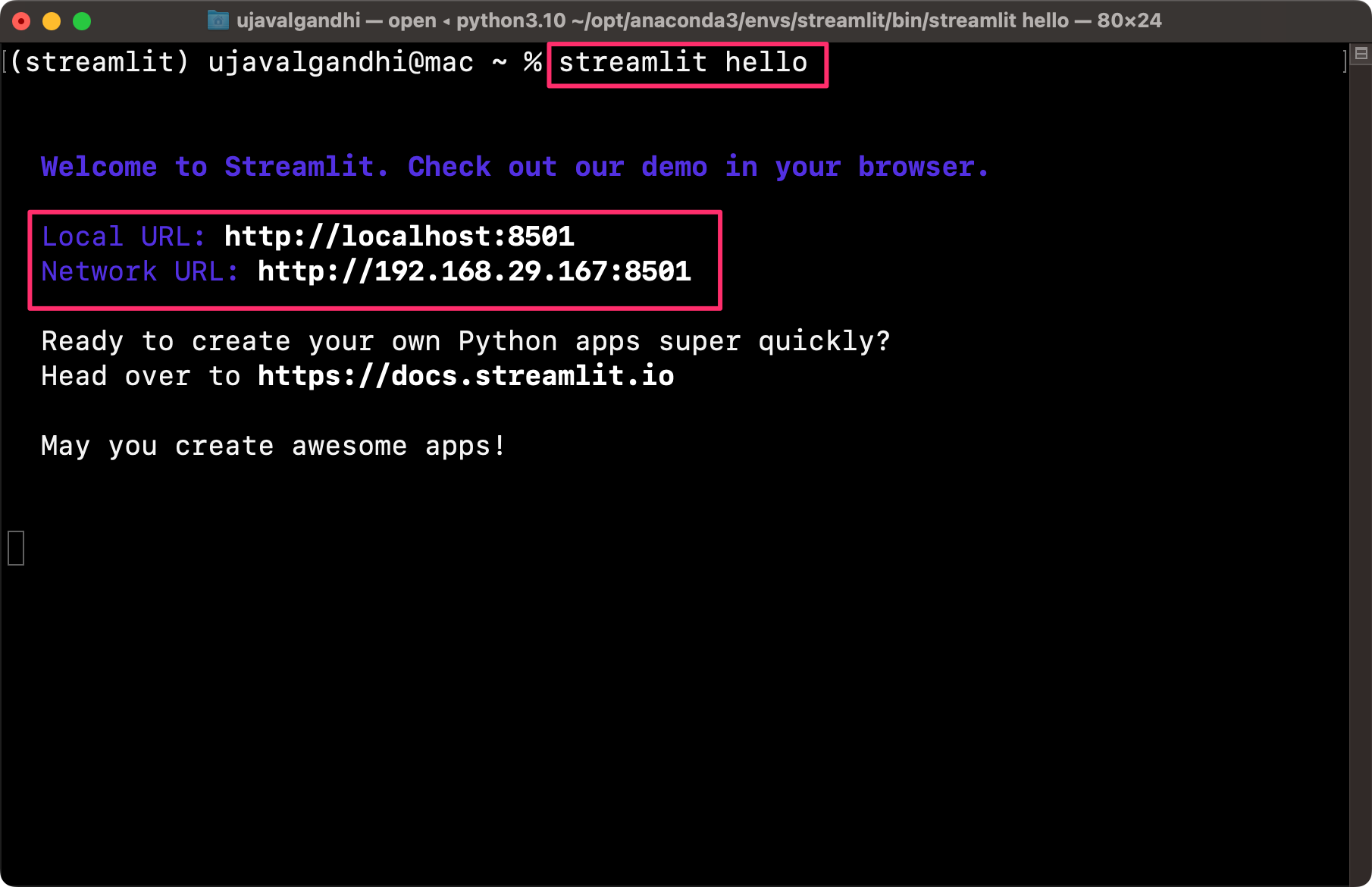A new browser tab will open and display the streamlit Hello app.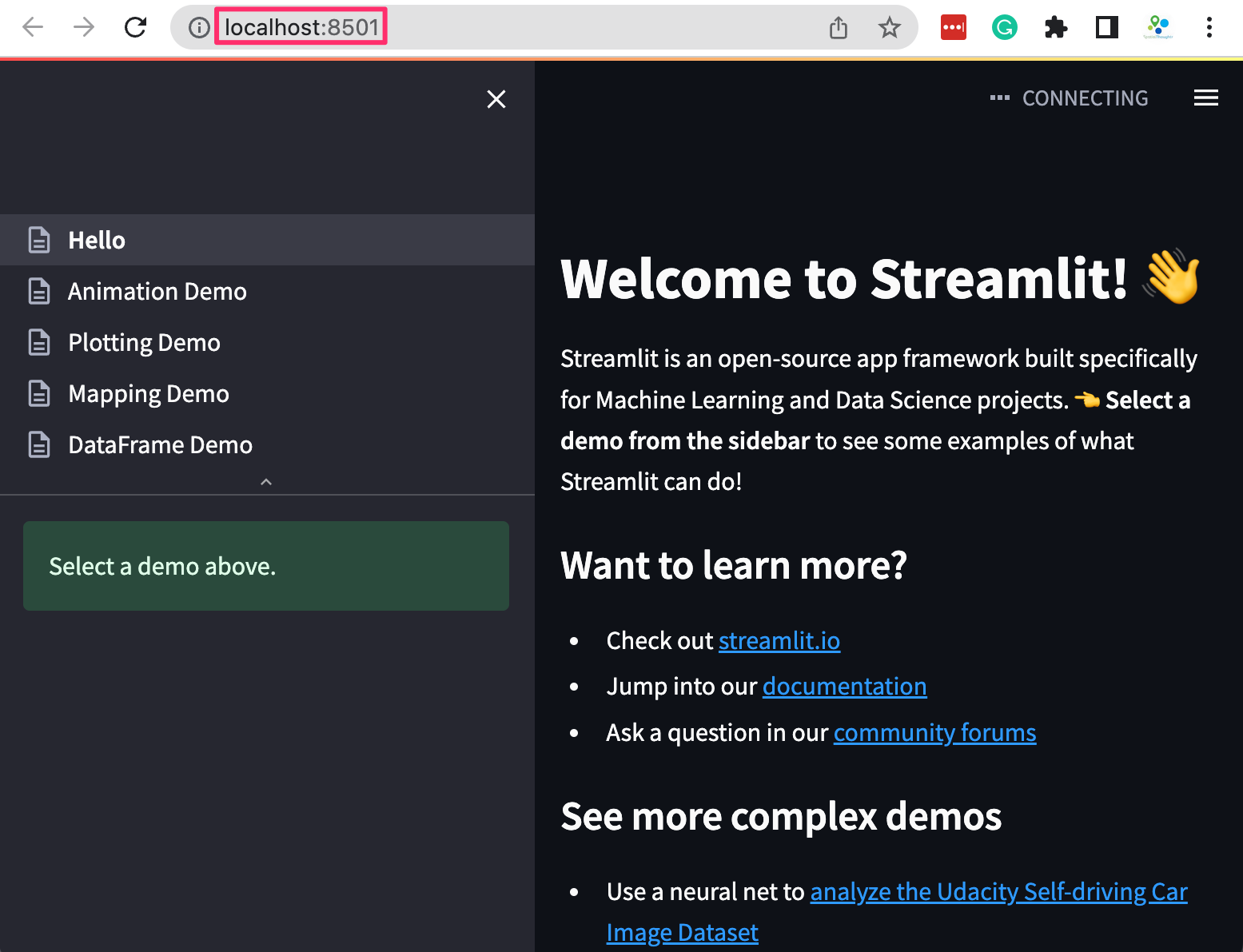Your installation is now complete. You can close the terminal window to stop the streamlit server.

## Create a Simple Dashboard

Let’s create a simple interactive dashboard by loading a CSV file and displaying a chart with some statistics. We will get familiar with the streamlit app development workflow and explore different widgets and layout elements.

1. Create a folder named simple_dashboard on your Desktop.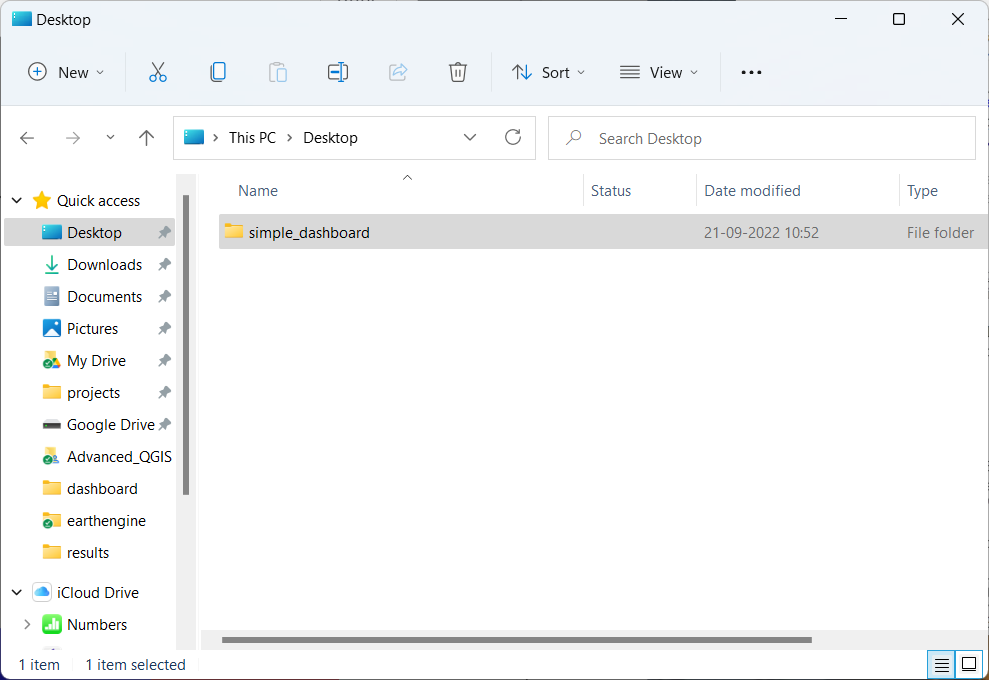1. Open your favorite text editor and create a file with the following content. By convention, we import streamlit as st. Then we can use the st.title() to display the title of our dashboard and st.write() to add a paragraph of text. Save the file in the simple_dashboard folder on your desktop as app.py
import streamlit as st

st.title('A Simple Dashboard')
st.write('This dashboard displays a chart for the selected region.')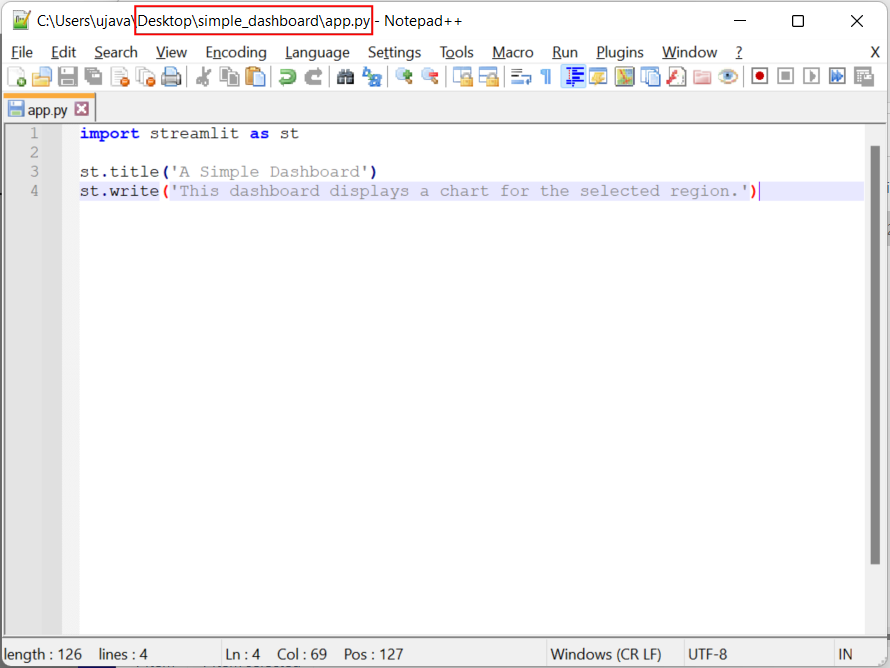1. Once the file is saved, open Anaconda Prompt (Windows) or Terminal (Mac/Linux). Switch to the conda environment where you have installed the required packages. We then use cd command to change the current directory to the once with the app.py file. Then run the following command to start the streamlit server and launch the app.
streamlit run app.py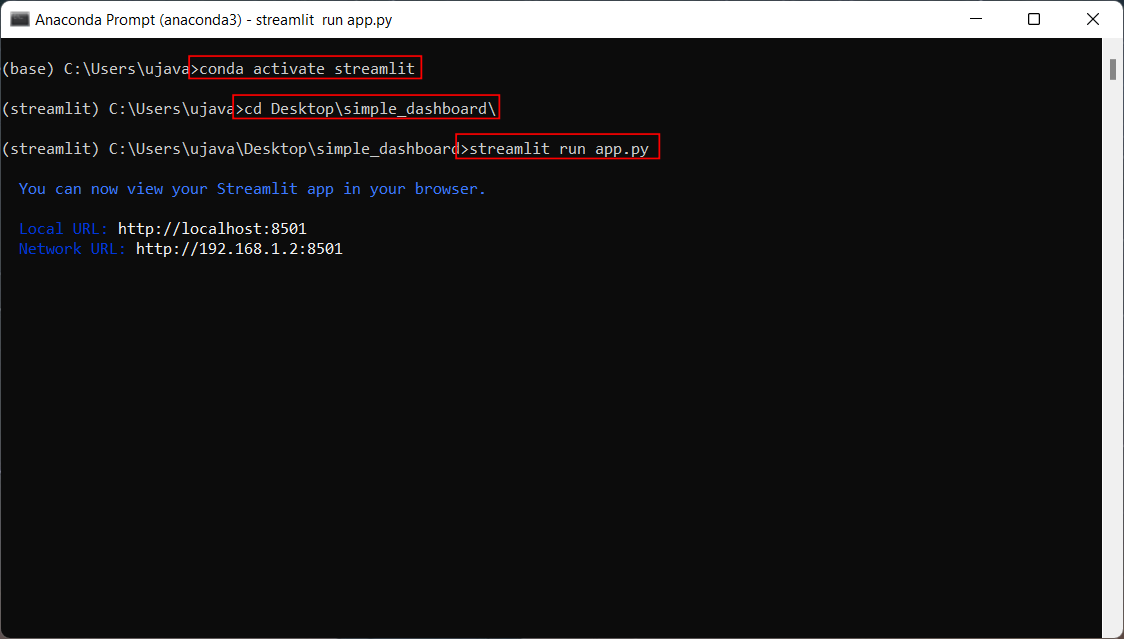1. A new browser tab will open and display the output of the app.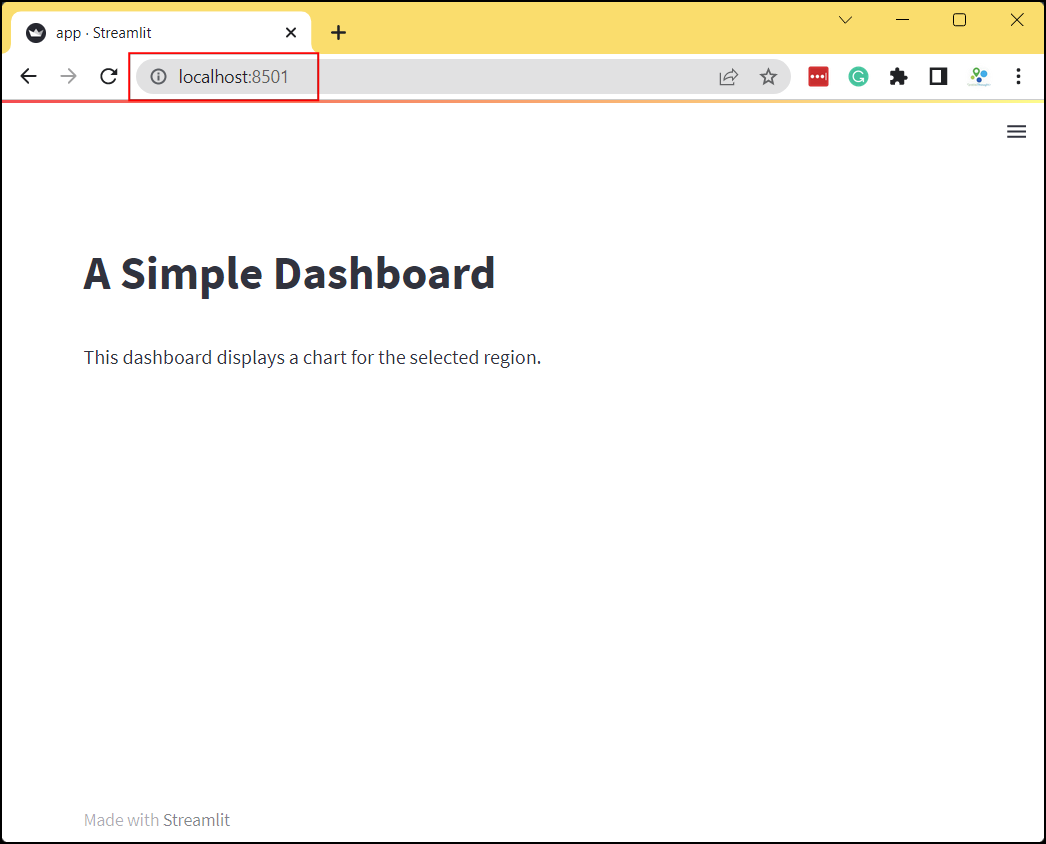1. Let’s read some data and display it. We load a CSV file from a URL using Pandas and get a DataFrame object. We then use st.dataframe() widget to render the dataframe. Update your app.py with the following code. As you save the file, you will notice that streamlit will detect it and display a prompt to re-run your app. Choose Always rerun.
import streamlit as st
import pandas as pd

st.title('A Simple Dashboard')
st.write('This dashboard displays a chart for the selected region.')

csv_file = 'highway_lengths_by_district.csv'

url = data_url + csv_file

st.dataframe(df)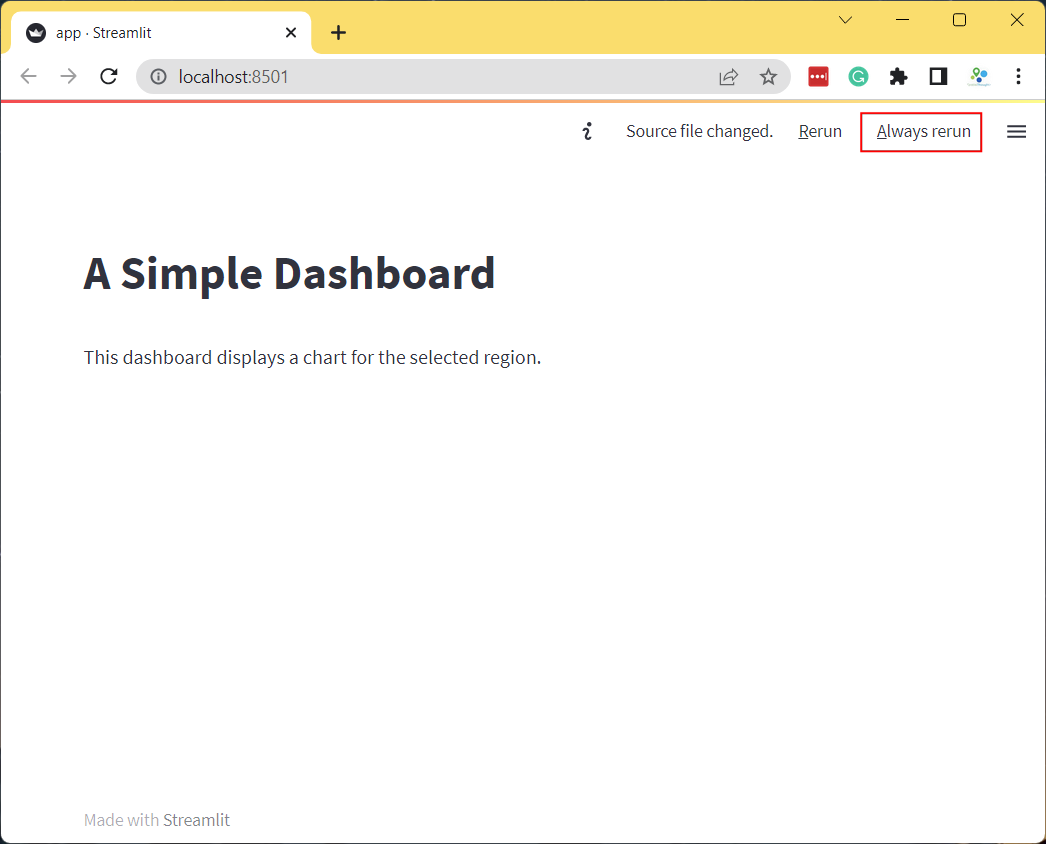1. The app will now display the dataframe. The dataframe consists of 3 columns. The DISTRICT column contains a unique name for the admin region. The NH and SH columns contain the length of National Highways and State Highways in Kilometers for each admin region. We will now create a dashboard that displays a chart with the lengths of highways for the user-selected admin region.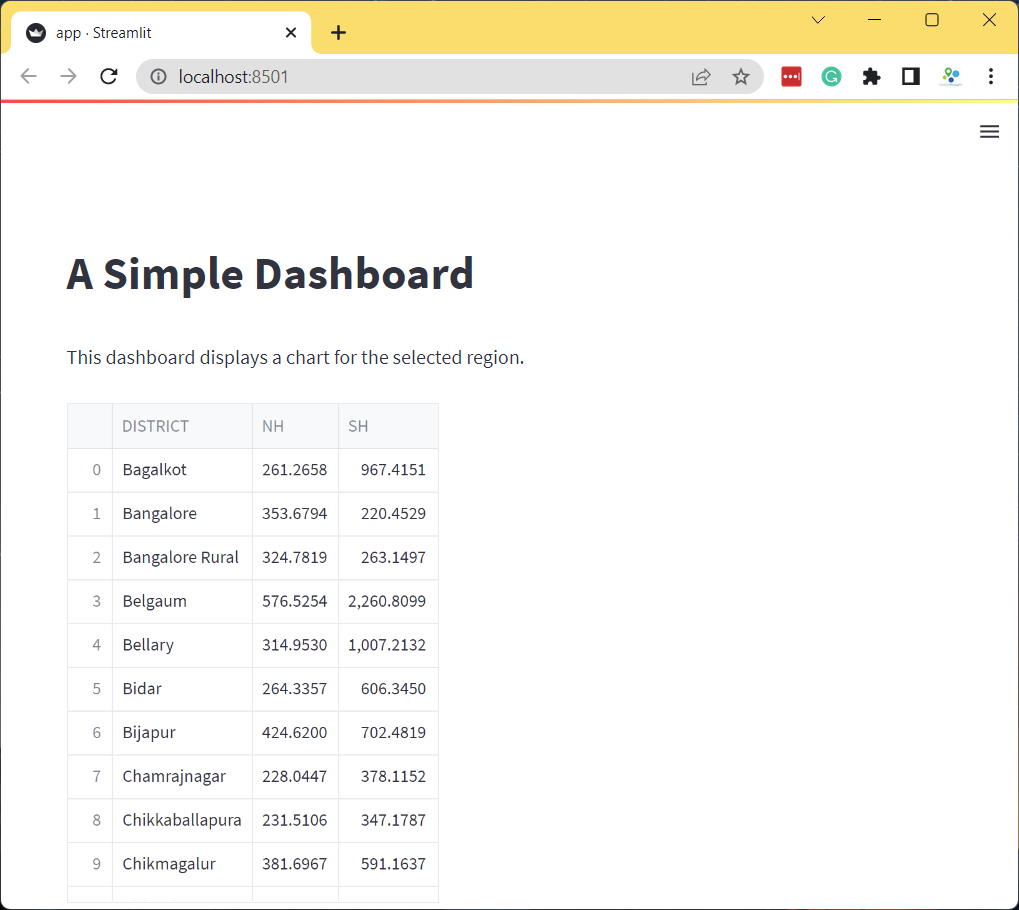1. Let’s add a dropdown menu with the list of admin regions. We first get the names from the DISTRICT column and use st.selectbox() to add a dropdown selector. Streamlit apps always run the entire app.py whenever any selection is changed. With our current app structure, whenever the user selected a new admin region, the source file will be fetched again and a new dataframe will be created. This is not required as the source data does not change on every interaction. A good practice is to put the data fetching in a function and use the @st.cache_data decorator which will cache the results. Anytime the function is called with the same arguments, it will return the cached version of the data instead of fetching it.
import streamlit as st
import pandas as pd

st.title('A Simple Dashboard')
st.write('This dashboard displays a chart for the selected region.')

@st.cache_data
csv_file = 'highway_lengths_by_district.csv'

url = data_url + csv_file

return df

districts = df.DISTRICT.values
district = st.selectbox('Select a district', districts)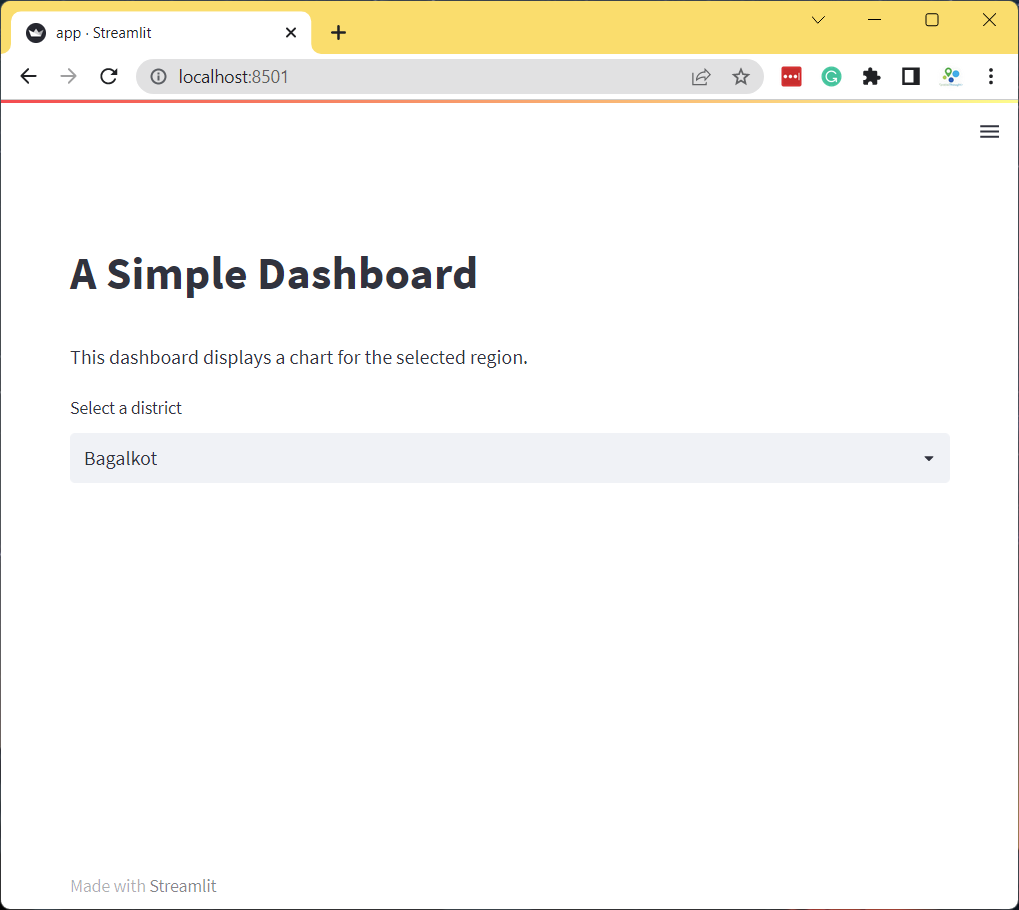1. As the user selects an admin region from the selectbox, the selected value is saved in the district variable. We use it to filter the DataFrame to the selected district. Then we use Matplotlib to create a bar-chart with the filtered dataframe and display it using st.pyplot() widget.
import streamlit as st
import pandas as pd
import matplotlib.pyplot as plt

st.title('A Simple Dashboard')
st.write('This dashboard displays a chart for the selected region.')

@st.cache_data
csv_file = 'highway_lengths_by_district.csv'

url = data_url + csv_file

return df

districts = df.DISTRICT.values
district = st.selectbox('Select a district', districts)
filtered = df[df['DISTRICT'] == district]

fig, ax = plt.subplots(1, 1)

filtered.plot(kind='bar', ax=ax, color=['#0000FF', '#FF0000'],
ylabel='Kilometers', xlabel='Category')
ax.set_xticklabels([])
stats = st.pyplot(fig)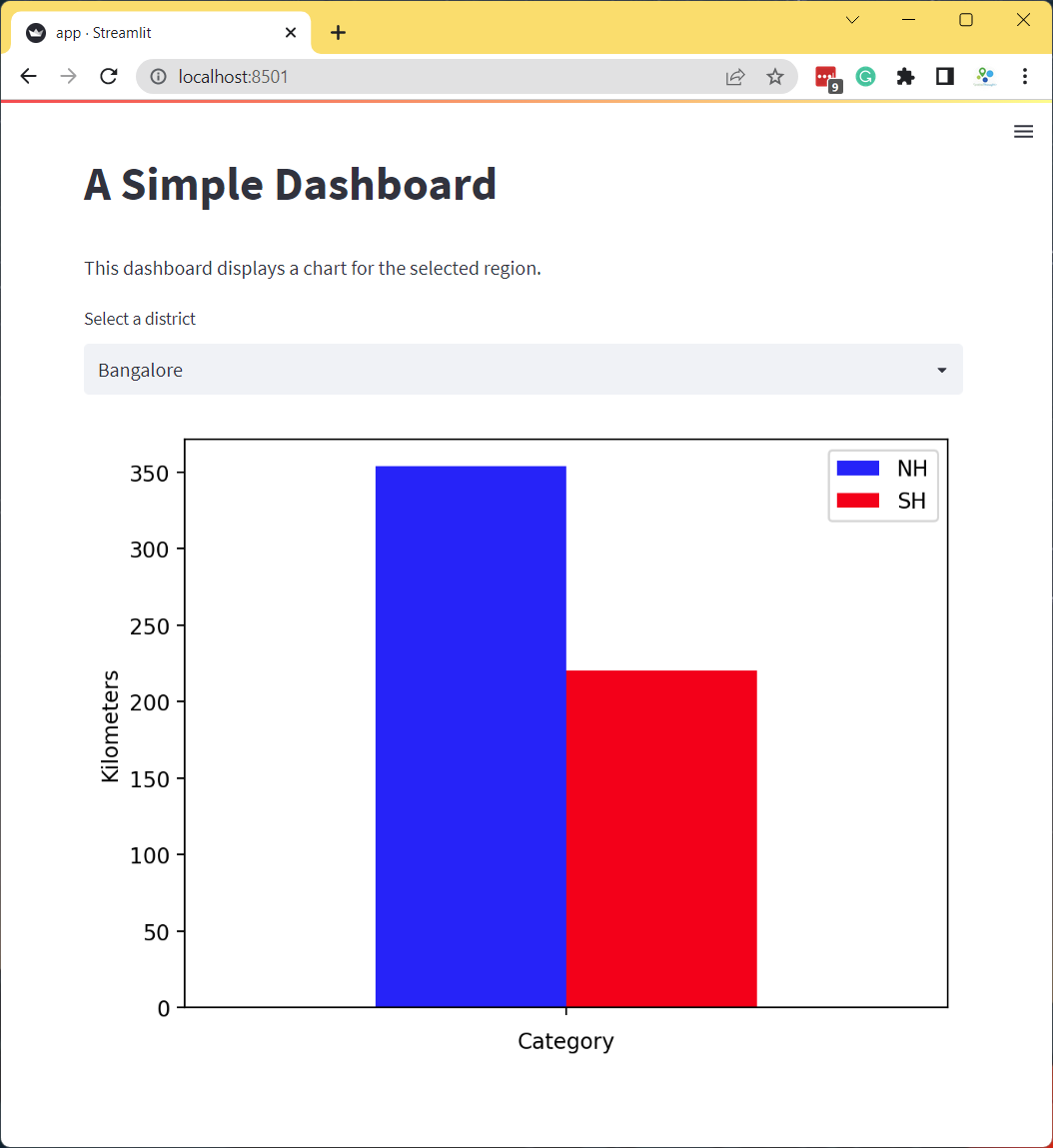1. Our dashboard now displays an updated chart every-time you change the selection. Streamlit provides many other user-interface widgets. Let’s explore some more of them. We will use st.color_picker() widget to allow users to customize the color of the bar chart. We can display 2 color-pickers side-by-side using a column layout. We use st.columns() to create 2 columns col1 and col2. We add a color_picker() to each column with appropriate label and a default color. If we have more than 1 widget of the same type in the app, we need to provide a unique key that can be used to identify the widget. We make some final tweaks to the chart and complete the dashboard.
import streamlit as st
import pandas as pd
import matplotlib.pyplot as plt

st.title('A Simple Dashboard')
st.write('This dashboard displays a chart for the selected region.')

@st.cache_data
csv_file = 'highway_lengths_by_district.csv'

url = data_url + csv_file

return df

districts = df.DISTRICT.values
district = st.selectbox('Select a district', districts)
filtered = df[df['DISTRICT'] == district]

col1, col2 = st.columns(2)

nh_color = col1.color_picker('Pick NH Color', '#0000FF', key='nh')
sh_color = col2.color_picker('Pick SH Color', '#FF0000', key='sh')

fig, ax = plt.subplots(1, 1)

filtered.plot(kind='bar', ax=ax, color=[nh_color, sh_color],
ylabel='Kilometers', xlabel='Category')
ax.set_title('Length of Highways')
ax.set_ylim(0, 2500)
ax.set_xticklabels([])
stats = st.pyplot(fig)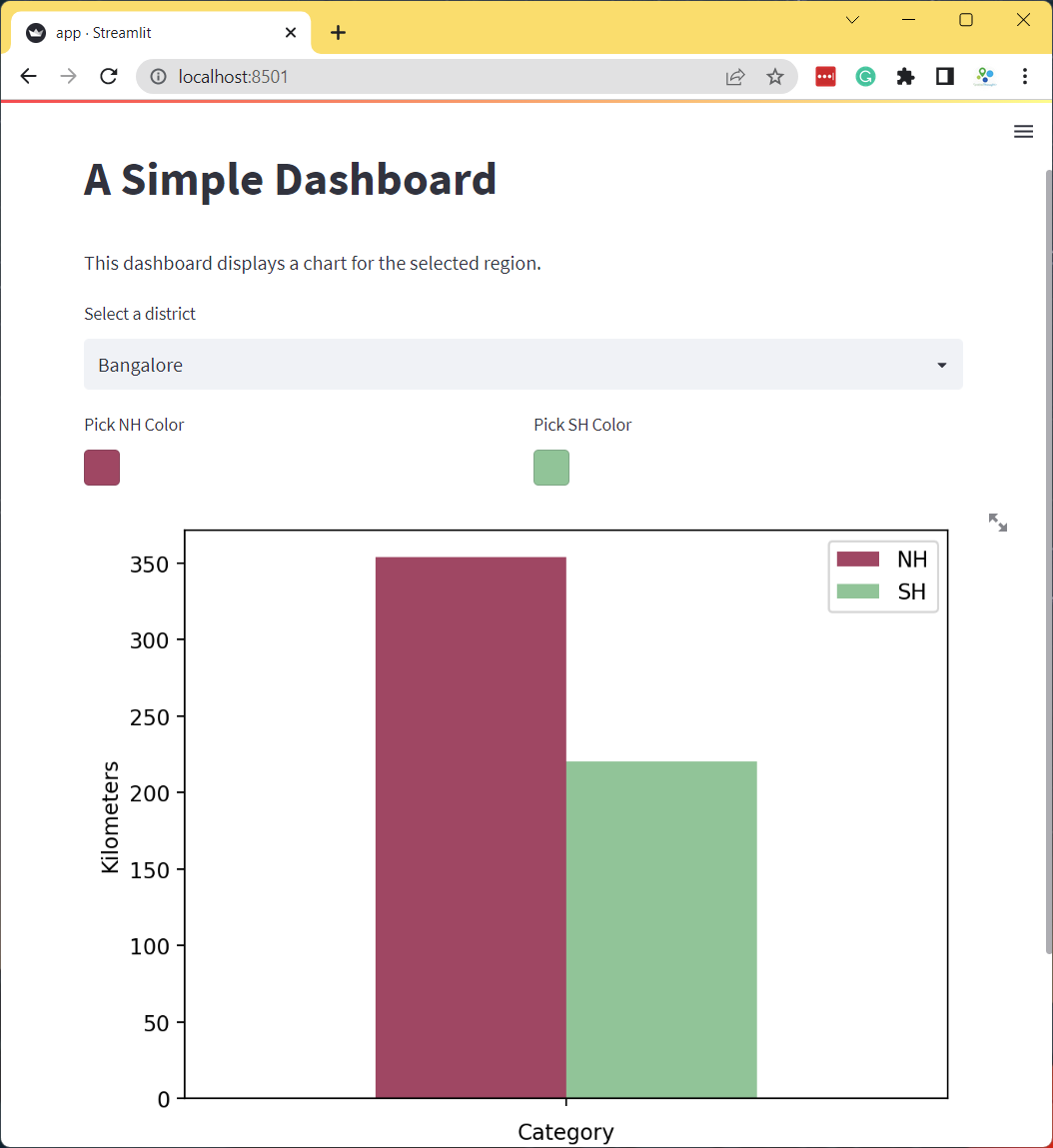## Exercise

Add a radio-button to the app that allows the user to select the units for length between Kilometers and Miles as shown below. As the user toggles the radio-button, you should apply the appropriate conversion from Kilometer to Miles and update the chart.

• Hint1: Change st.columns() to have 3 columns and save the results into 3 separate objects. col1, col2, col3 = st.columns(3)
• Hint2: You can convert the values from Kilometer to Miles using filtered = filtered[['NH', 'SH']]*0.621371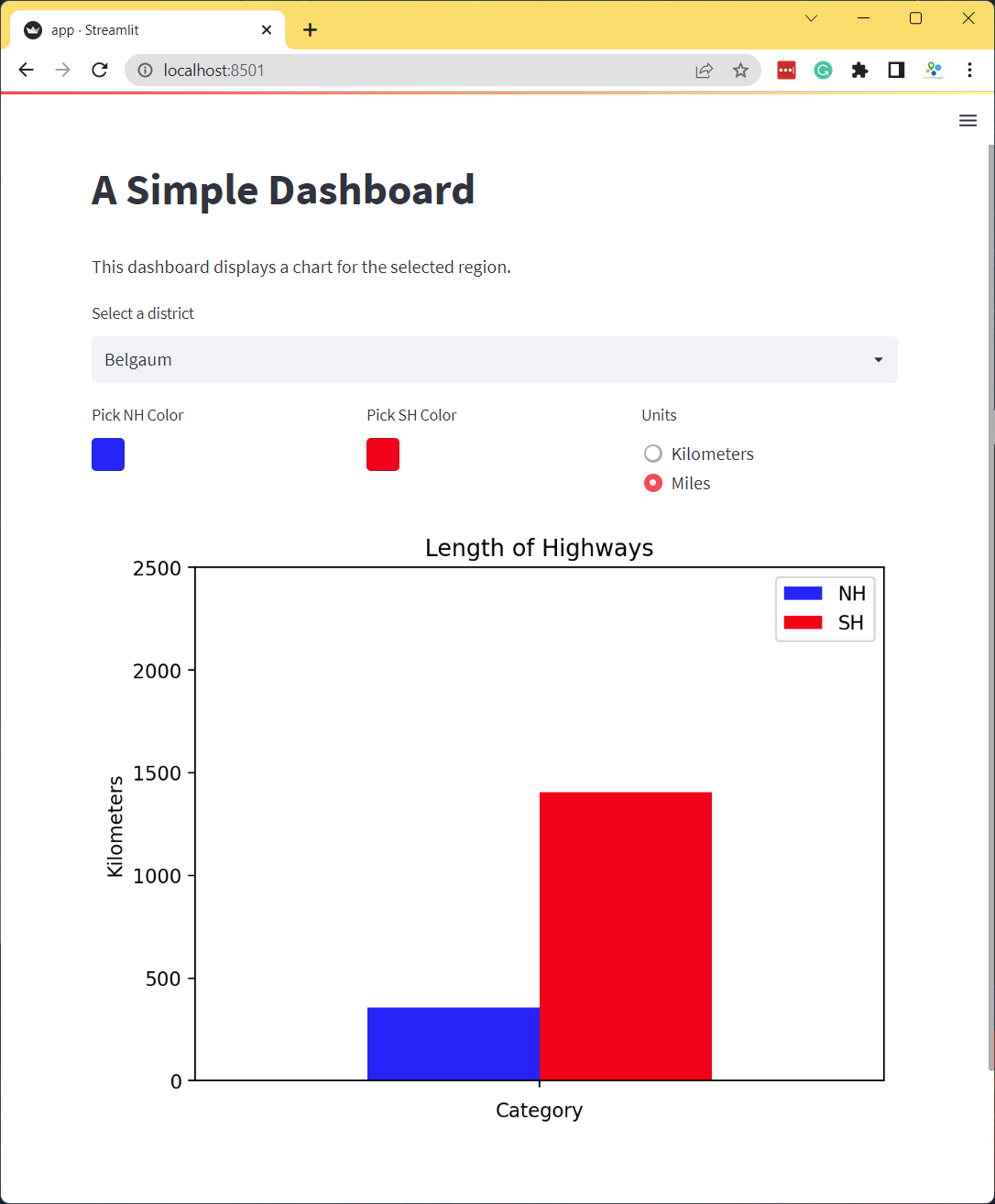## Create a Simple App

Let’s create a simple app that geocodes a user query and displays the results on a map. We will use OpenRouteService Geocoding API for geocoding and Folium to display the results on a map.

1. We start by creating a new folder simple_app and create the app.py with a basic layout with a title, a description and a text input for the address.
import folium
import requests
import streamlit as st

st.title('A Simple Geocoder')
st.markdown('This app uses the [OpenRouteService API](https://openrouteservice.org/) '
'to geocode the input address and display the results on a map.')

address = st.text_input('Enter an address.')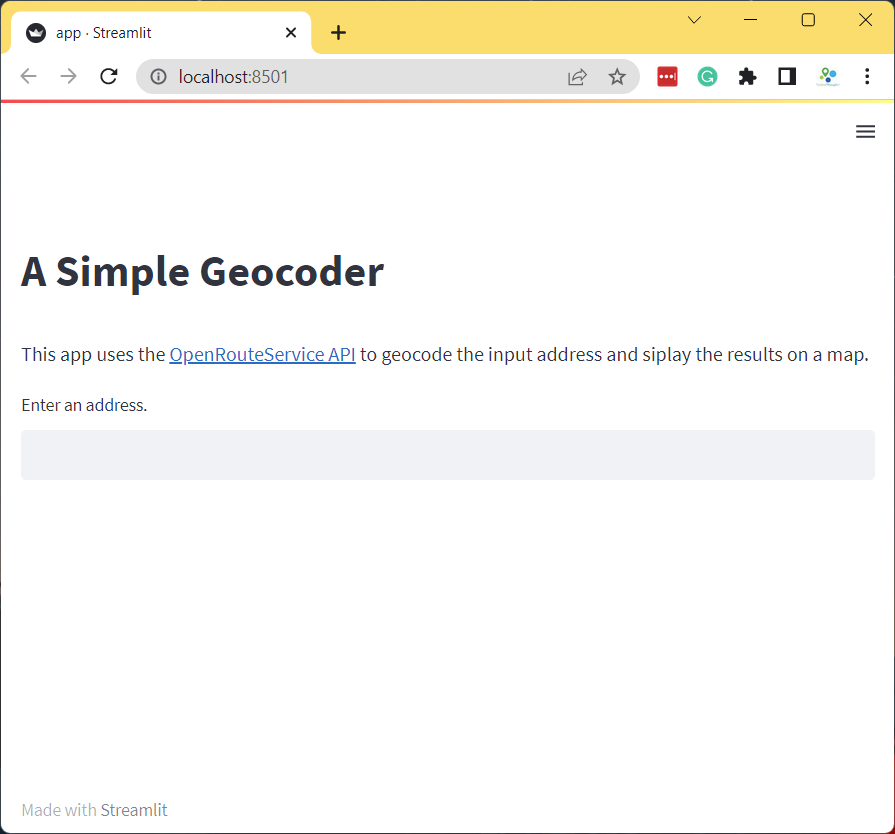1. Now we add a geocode() function that will take an address and geocode it using OpenRouteService API.
import requests
import streamlit as st

st.title('A Simple Geocoder')
st.markdown('This app uses the [OpenRouteService API](https://openrouteservice.org/) '
'to geocode the input address and display the results on a map.')

@st.cache_data
def geocode(query):
parameters = {
'api_key': ORS_API_KEY,
'text' : query
}

response = requests.get(
'https://api.openrouteservice.org/geocode/search',
params=parameters)
if response.status_code == 200:
data = response.json()
if data['features']:
x, y = data['features']['geometry']['coordinates']
return (y, x)

if results:
st.write('Geocoded Coordinates: {}, {}'.format(results, results))
else:
st.error('Request failed. No results.')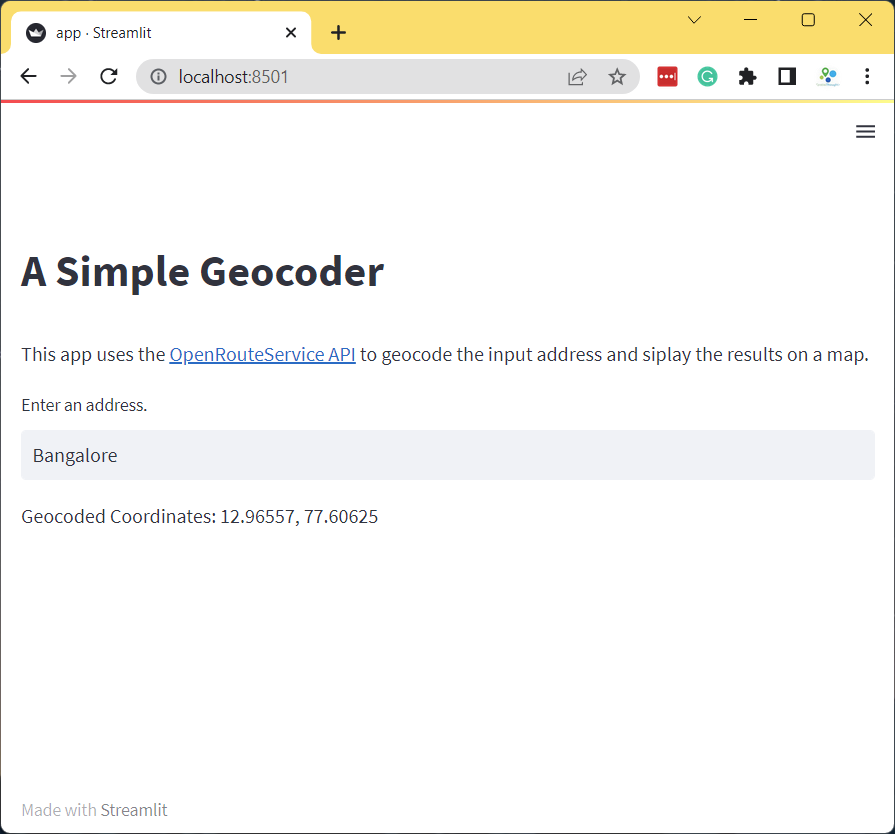1. Now that we have coordinates, we can display it on a map using the streamlit_folium component.
import folium
import requests
import streamlit as st
from streamlit_folium import folium_static

st.title('A Simple Geocoder')
st.markdown('This app uses the [OpenRouteService API](https://openrouteservice.org/) '
'to geocode the input address and siplay the results on a map.')

@st.cache_data
def geocode(query):
parameters = {
'api_key': ORS_API_KEY,
'text' : query
}

response = requests.get(
'https://api.openrouteservice.org/geocode/search',
params=parameters)
if response.status_code == 200:
data = response.json()
if data['features']:
x, y = data['features']['geometry']['coordinates']
return (y, x)

if results:
st.write('Geocoded Coordinates: {}, {}'.format(results, results))

m = folium.Map(location=results, zoom_start=8)
folium.Marker(
results,
icon=folium.Icon(color='green', icon='crosshairs', prefix='fa')
folium_static(m, width=800)

else:
st.error('Request failed. No results.')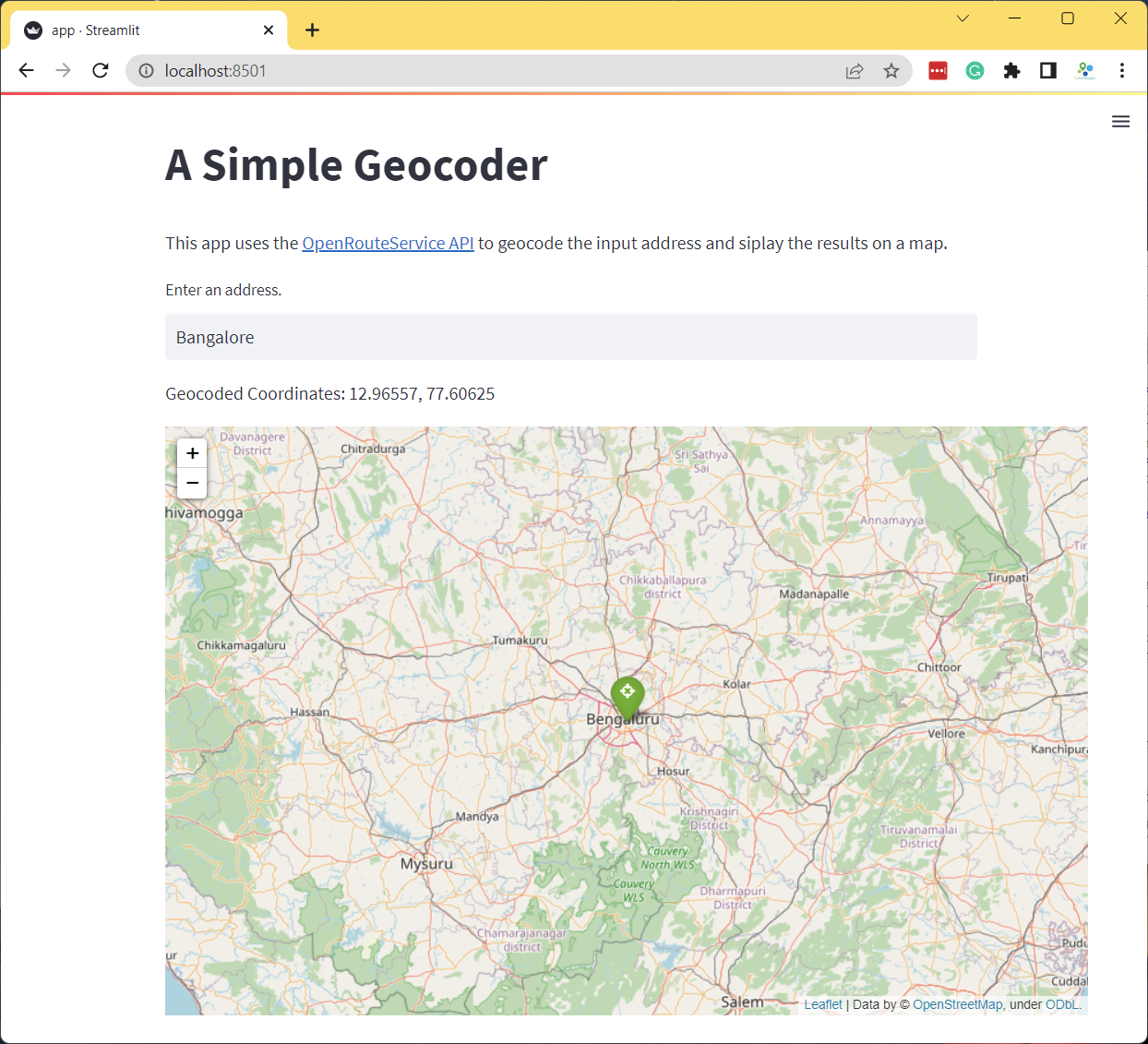## Exercise

Add a dropdown menu to give the users an option to change the default basemap of the Folium map.

• Hint: Add a st.selectbox() with basemap strings. st.selectbox('Select a basemap', ['OpenStreetMap', 'Stamen Terrain', 'Stamen Toner'])

Reference: folium.folium.Map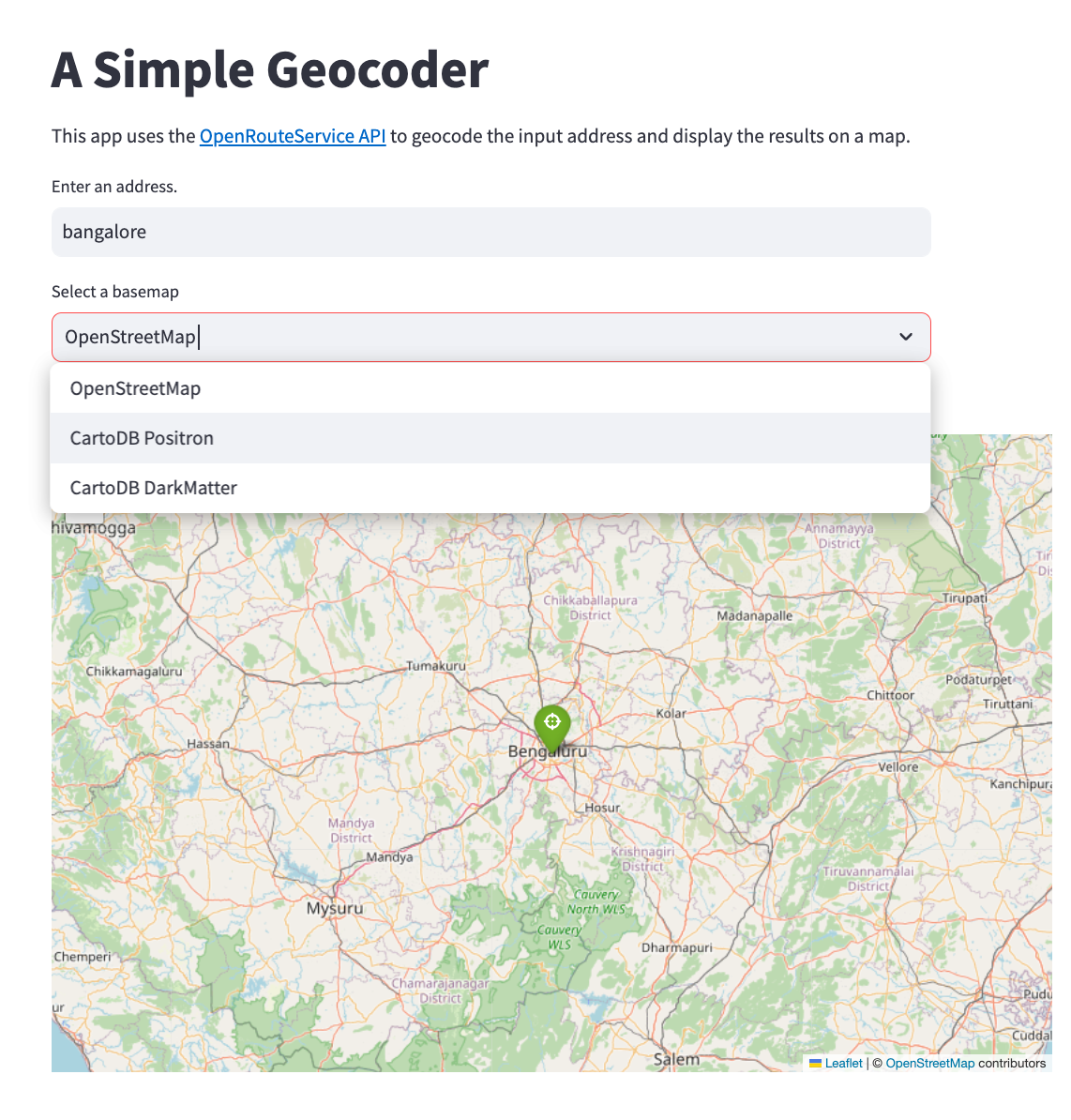# Building Mapping Apps with Leafmap and Streamlit

We can leverag leafmap to create an interactive mapping dashboard that gives us the flexibility of using many different mapping backends and way to read a wide-variety of spatial data formats.

## Create a Mapping Dashboard

The code below creates an interactive mapping dashboard that displays the statistics of the selected region.

1. We start by creating the app directory mapping_dashboard and creating app.py with the following content. This code creates a layout with a sidebar using st.sidebar() and adds some widgets to it. Note that while we need to use st.title() for the main section, we use st.sidebar.title() for the sidebar.
import streamlit as st

st.set_page_config(page_title='Dashboard', layout='wide')

st.title('Highway Dashboard')

st.sidebar.info('Explore the Highway Statistics')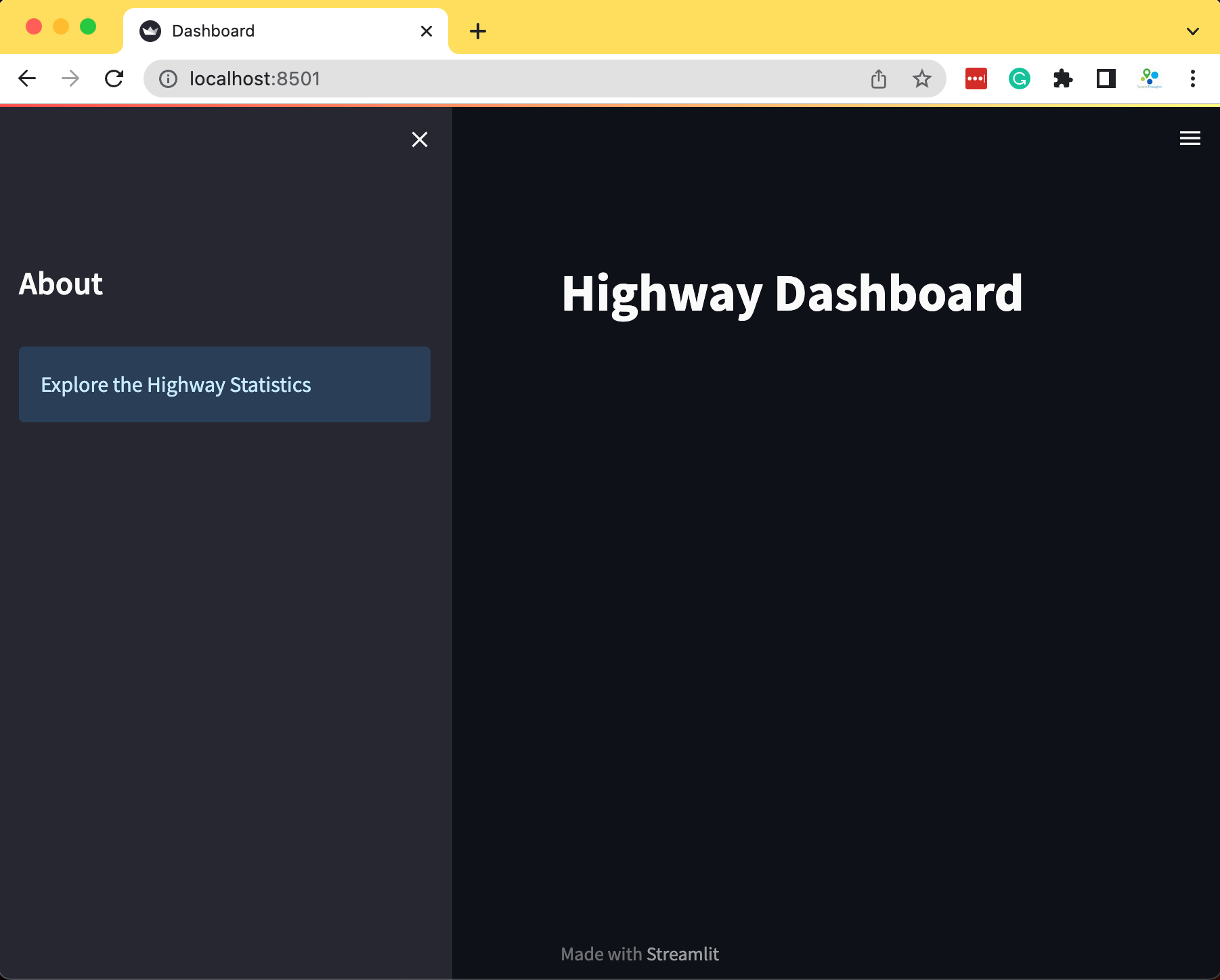1. We now use geopandas to read 2 vector layers from a geopackage and pandas to read a CSV file containing road statistics. We put the code for data fetching inside a function and cache it using the st.cache_data decorator.
import streamlit as st
import geopandas as gpd
import pandas as pd

st.set_page_config(page_title='Dashboard', layout='wide')

st.title('Highway Dashboard')

st.sidebar.info('Explore the Highway Statistics')

gpkg_file = 'karnataka.gpkg'
csv_file = 'highway_lengths_by_district.csv'

@st.cache_data
return gdf

@st.cache_data
return df

gpkg_url = data_url + gpkg_file
csv_url = data_url + csv_file
data_load_state.text('Loading data... done!')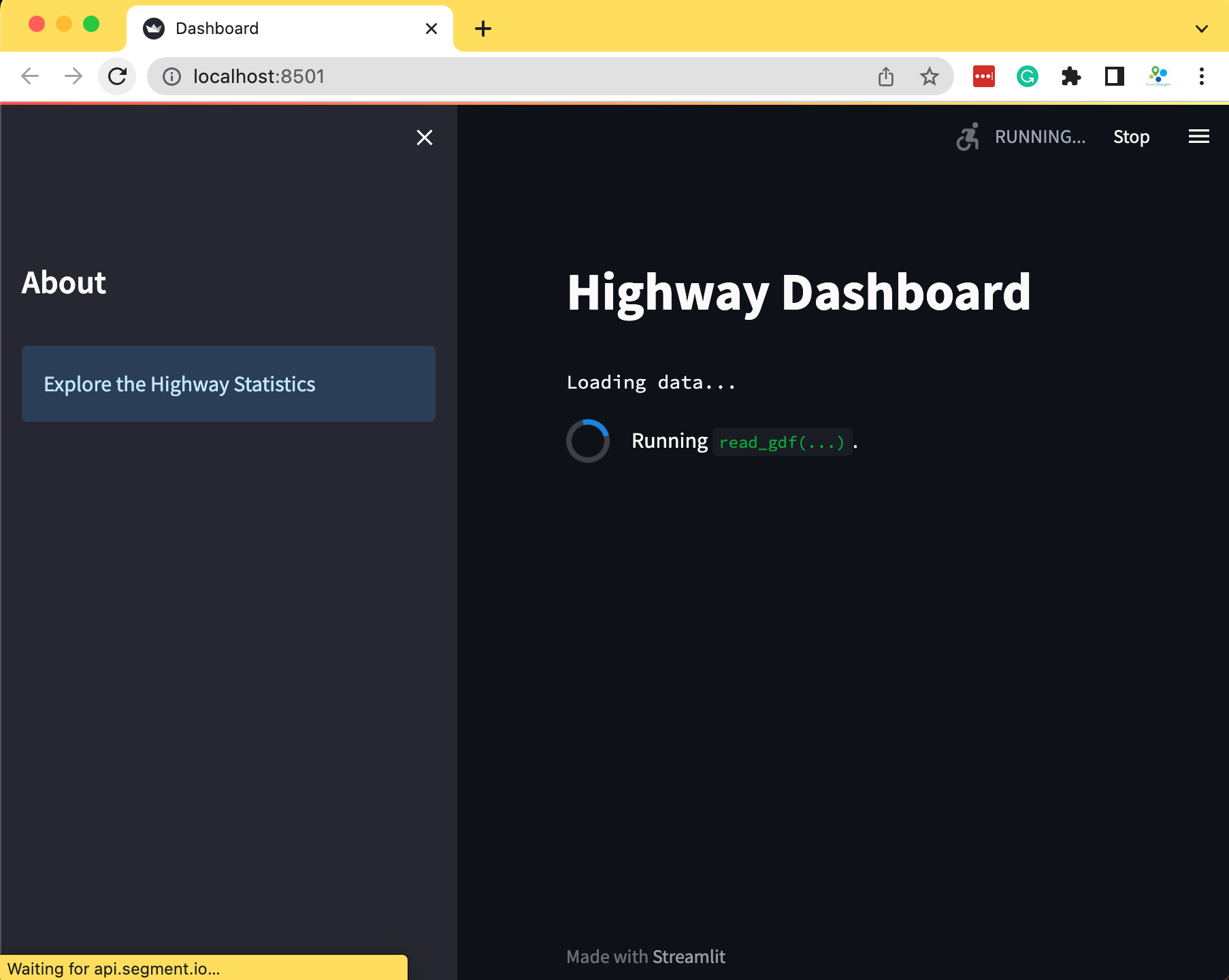1. Now we use the information in the CSV file to display a chart in the sidebar.
import streamlit as st
import geopandas as gpd
import pandas as pd
import matplotlib.pyplot as plt

st.set_page_config(page_title='Dashboard', layout='wide')

st.title('Highway Dashboard')

st.sidebar.info('Explore the Highway Statistics')

gpkg_file = 'karnataka.gpkg'
csv_file = 'highway_lengths_by_district.csv'

@st.cache_data
return gdf

@st.cache_data
return df

gpkg_url = data_url + gpkg_file
csv_url = data_url + csv_file

# Create the chart
districts = districts_gdf.DISTRICT.values
district = st.sidebar.selectbox('Select a District', districts)

district_lengths = lengths_df[lengths_df['DISTRICT'] == district]

fig, ax = plt.subplots(1, 1)
district_lengths.plot(kind='bar', ax=ax, color=['blue', 'red'],
ylabel='Kilometers', xlabel='Category')
ax.set_xticklabels([])
stats = st.sidebar.pyplot(fig)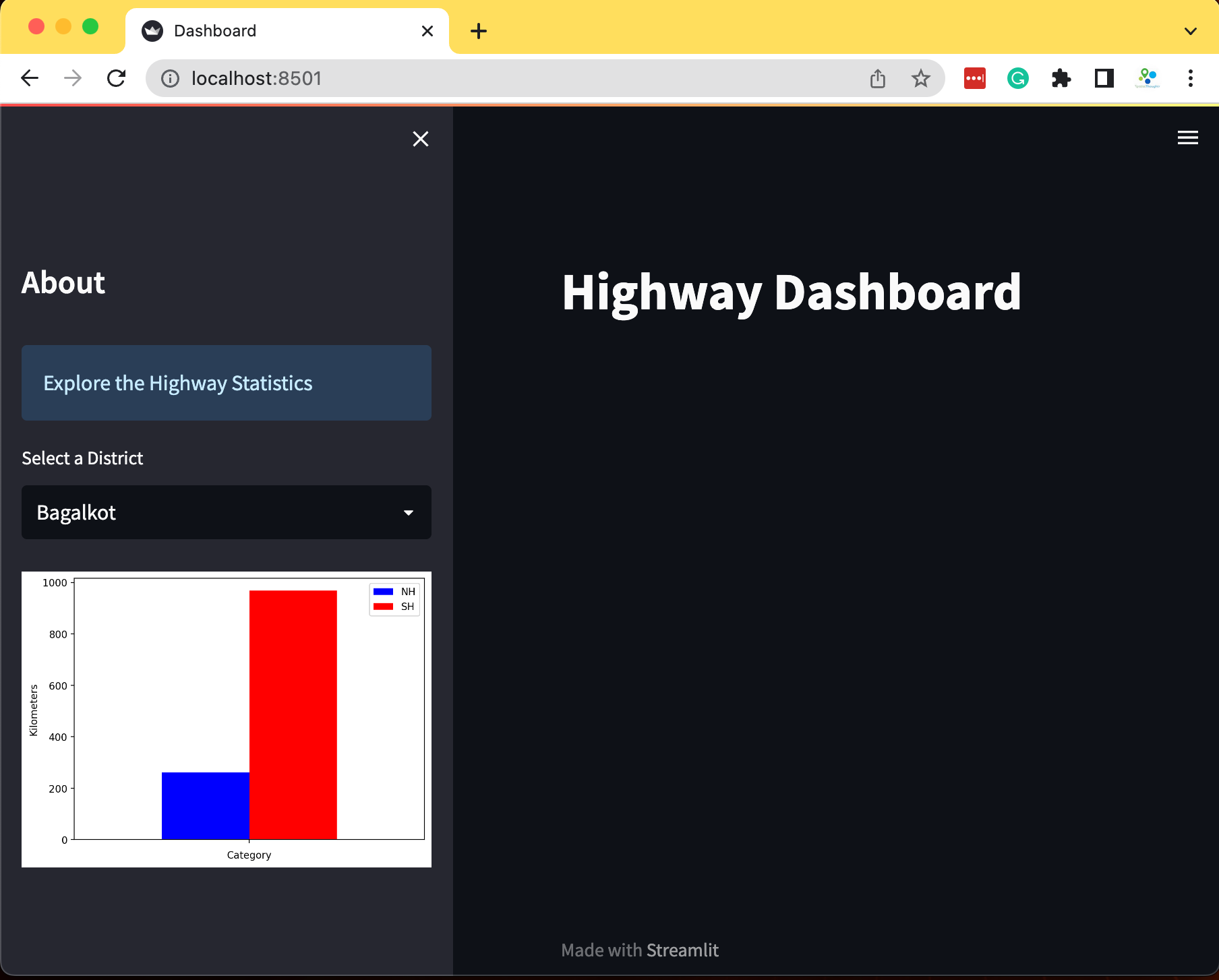1. Now we create a folium map using leafmap.Map() and render the vector layers. We also add a third layer with the boundary of the selected district to highlight the selection.
import streamlit as st
import geopandas as gpd
import pandas as pd
import matplotlib.pyplot as plt
import leafmap.foliumap as leafmap

st.set_page_config(page_title='Dashboard', layout='wide')

st.title('Highway Dashboard')

st.sidebar.info('Explore the Highway Statistics')

gpkg_file = 'karnataka.gpkg'
csv_file = 'highway_lengths_by_district.csv'

@st.cache_data
return gdf

@st.cache_data
return df

gpkg_url = data_url + gpkg_file
csv_url = data_url + csv_file

# Create the chart
districts = districts_gdf.DISTRICT.values
district = st.sidebar.selectbox('Select a District', districts)

district_lengths = lengths_df[lengths_df['DISTRICT'] == district]

fig, ax = plt.subplots(1, 1)
district_lengths.plot(kind='bar', ax=ax, color=['blue', 'red'],
ylabel='Kilometers', xlabel='Category')
ax.set_xticklabels([])
stats = st.sidebar.pyplot(fig)

## Create the map

m = leafmap.Map(
layers_control=True,
draw_control=False,
measure_control=False,
fullscreen_control=False,
)
gdf=districts_gdf,
zoom_to_layer=False,
layer_name='districts',
info_mode='on_click',
style={'color': '#7fcdbb', 'fillOpacity': 0.3, 'weight': 0.5},
)

selected_gdf = districts_gdf[districts_gdf['DISTRICT'] == district]

gdf=selected_gdf,
layer_name='selected',
zoom_to_layer=True,
info_mode=None,
style={'color': 'yellow', 'fill': None, 'weight': 2}
)

m_streamlit = m.to_streamlit(600, 600)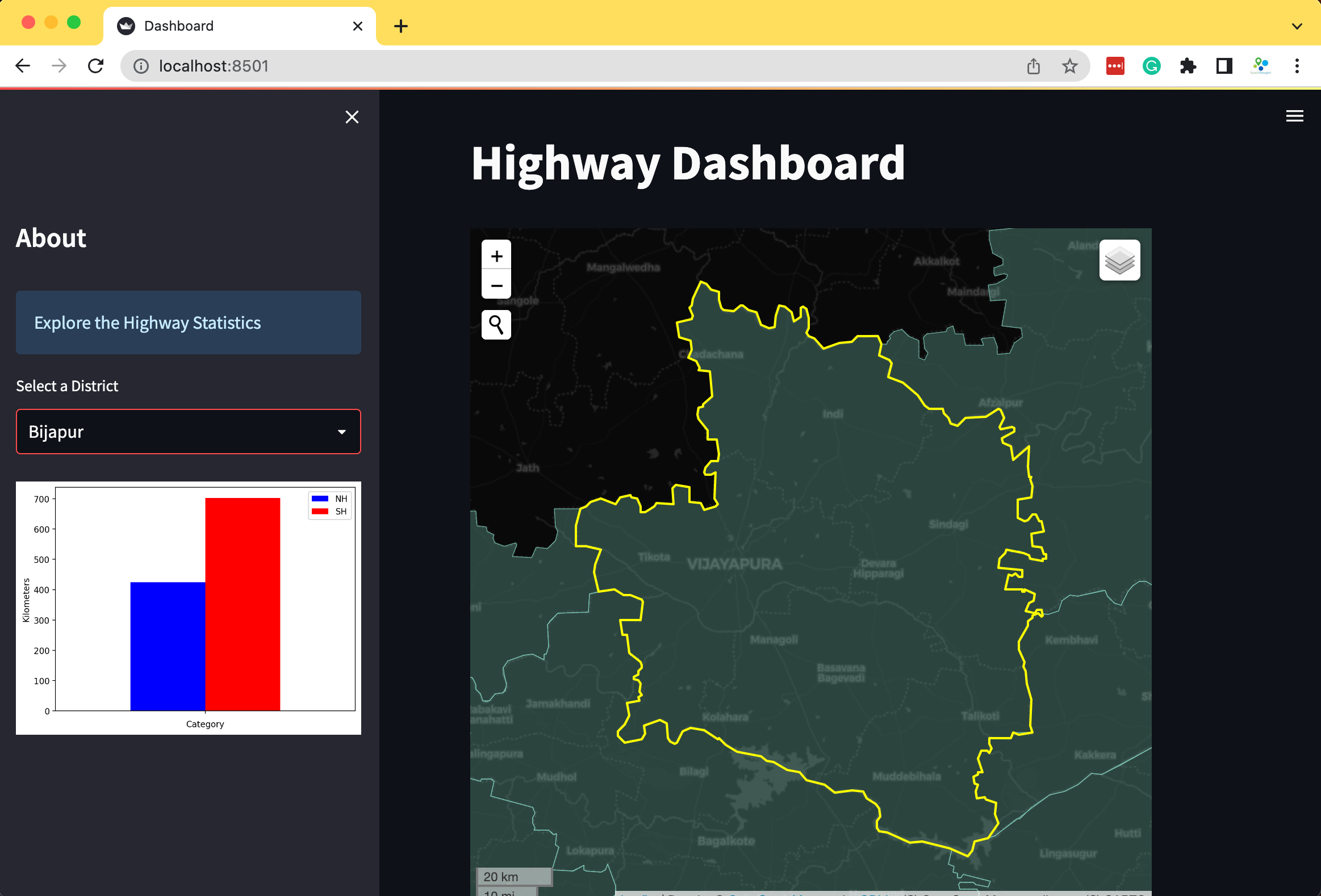1. We can selectively load certain layers on the map using a user-input widget. Let’s add a checkbox that allows the user to overlay the roads on the map.
import streamlit as st
import geopandas as gpd
import pandas as pd
import matplotlib.pyplot as plt
import leafmap.foliumap as leafmap

st.set_page_config(page_title='Dashboard', layout='wide')

st.title('Highway Dashboard')

st.sidebar.info('Explore the Highway Statistics')

gpkg_file = 'karnataka.gpkg'
csv_file = 'highway_lengths_by_district.csv'

@st.cache_data
return gdf

@st.cache_data
return df

gpkg_url = data_url + gpkg_file
csv_url = data_url + csv_file

# Create the chart
districts = districts_gdf.DISTRICT.values
district = st.sidebar.selectbox('Select a District', districts)
district_lengths = lengths_df[lengths_df['DISTRICT'] == district]

fig, ax = plt.subplots(1, 1)
district_lengths.plot(kind='bar', ax=ax, color=['blue', 'red'],
ylabel='Kilometers', xlabel='Category')
ax.get_xaxis().set_ticklabels([])
stats = st.sidebar.pyplot(fig)

## Create the map

m = leafmap.Map(
layers_control=True,
draw_control=False,
measure_control=False,
fullscreen_control=False,
)
gdf=districts_gdf,
zoom_to_layer=False,
layer_name='districts',
info_mode='on_click',
style={'color': '#7fcdbb', 'fillOpacity': 0.3, 'weight': 0.5},
)

if overlay:
zoom_to_layer=False,
layer_name='highways',
info_mode=None,
style={'color': '#225ea8', 'weight': 1.5},
)

selected_gdf = districts_gdf[districts_gdf['DISTRICT'] == district]

gdf=selected_gdf,
layer_name='selected',
zoom_to_layer=True,
info_mode=None,
style={'color': 'yellow', 'fill': None, 'weight': 2}
)

m_streamlit = m.to_streamlit(600, 600)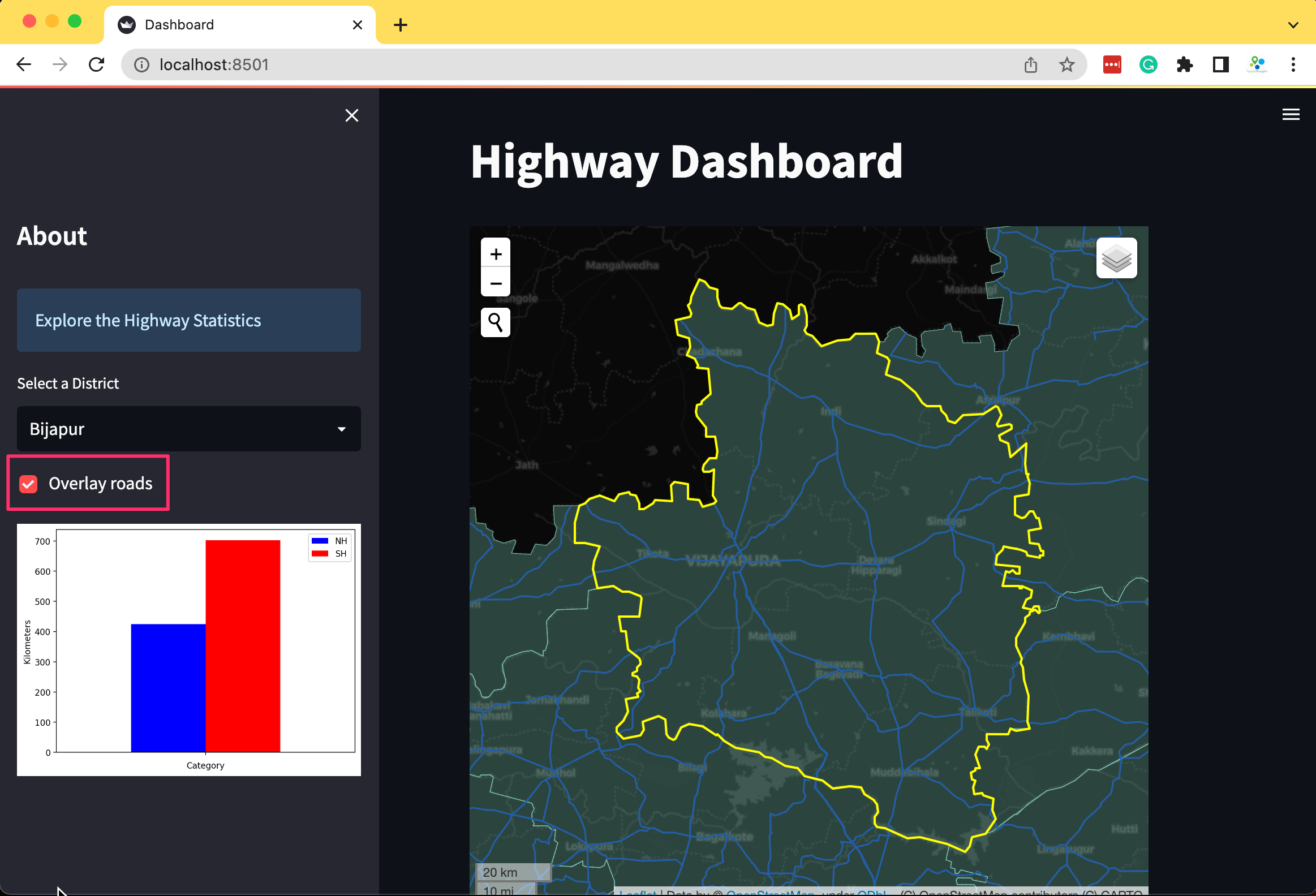# Publishing Apps with Streamlit Cloud

Streamlit provides free hosting for your streamlit apps. In this section, we will now learn how to deploy an app to Streamlit cloud and configure it correctly.

## Upload the app to GitHub

To run your app on Streamlit Cloud, you need to upload your app to GitHub. Streamlit supports both private and public repositories. In this example, we will be deploying a Route Finder app which has been uploaded to GitHub at https://github.com/spatialthoughts/python-dataviz-web/blob/main/streamlit/route_finder/app.py

If your app needs a third-party Python package, you need to add it in a separate file called requirements.txt. The packages listed in the file will be installed on Streamlit Cloud before running the app.

For our app, we have created the requirements.txt file with the following content and uploaded it to GitHub in the same directory as the app.py

streamlit
folium
streamlit-folium

## Replace Sensitive Data with Secrets

It is not a good practice to store API keys or passwords in the code as it can be seen by others and can be misused. Streamlit provides an easy way for Secrets Management. You can store any key=value pairs in a separate location and access it in the app using st.secrets.

While doing local development, you create a folder named .streamlit in the app directory and store any key=value pairs in a file named secrets.toml. For example, if you want to store the ORS API Key, you can create a new file secrets.toml in the .streamlit directory with the following content. (Replace <your api key> with the actual key)

'ORS_API_KEY' = '<your api key>'

Once done, the value of the ORS_API_KEY can be retrieved in the streamlit app using st.secrets['ORS_API_KEY']. While deploying the app, you can configure your secrets as outlined in the next section.

1. Visit Streamlit Cloud and sign-in. If you do not have an account, you can click Sign-up and create a new account. Once logged-in, click the New app button.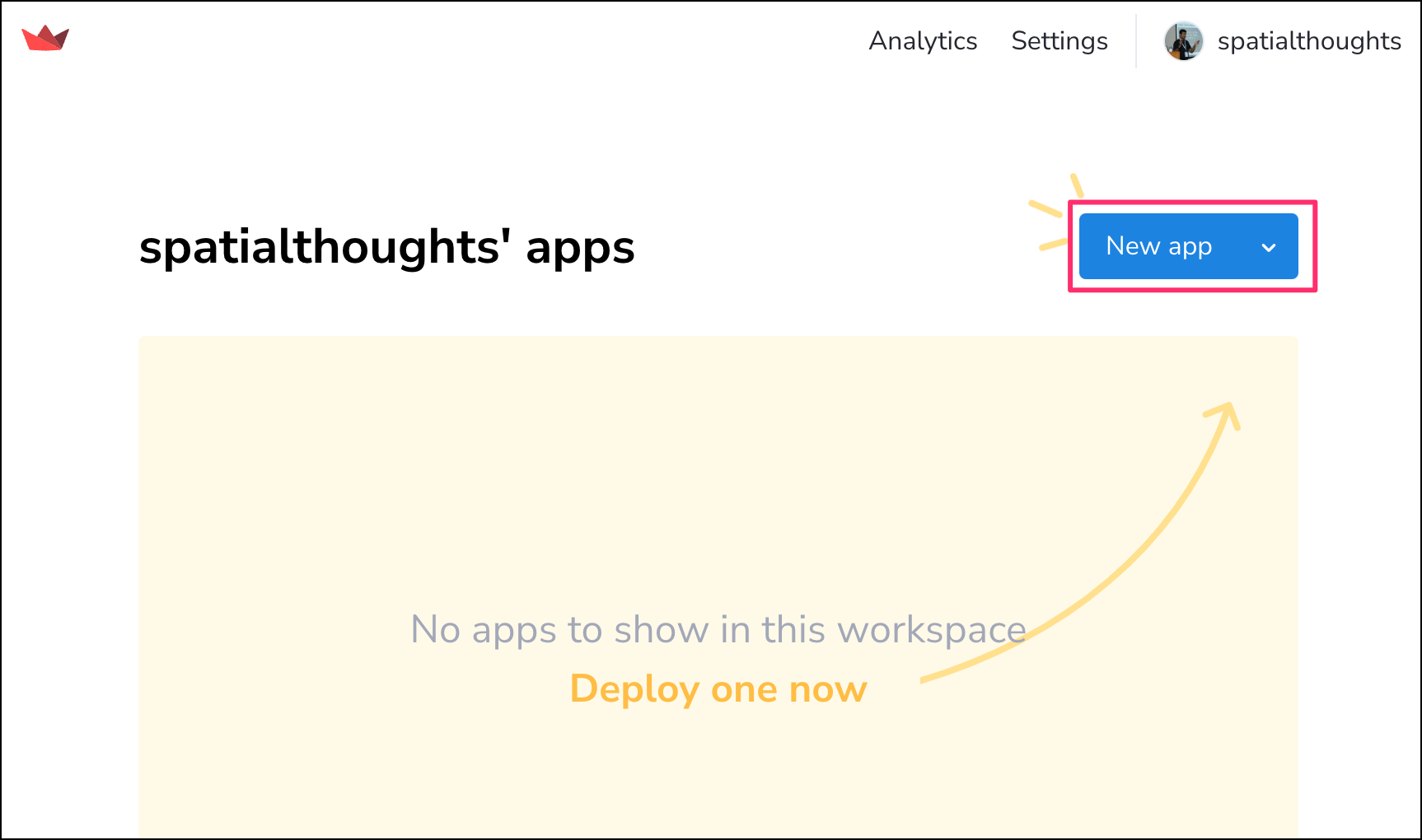1. Click Paste GitHub URL and paste the URL to your streamlit app.py file. For this example, you may use for the Route Finder app. Next, click Advanced settings…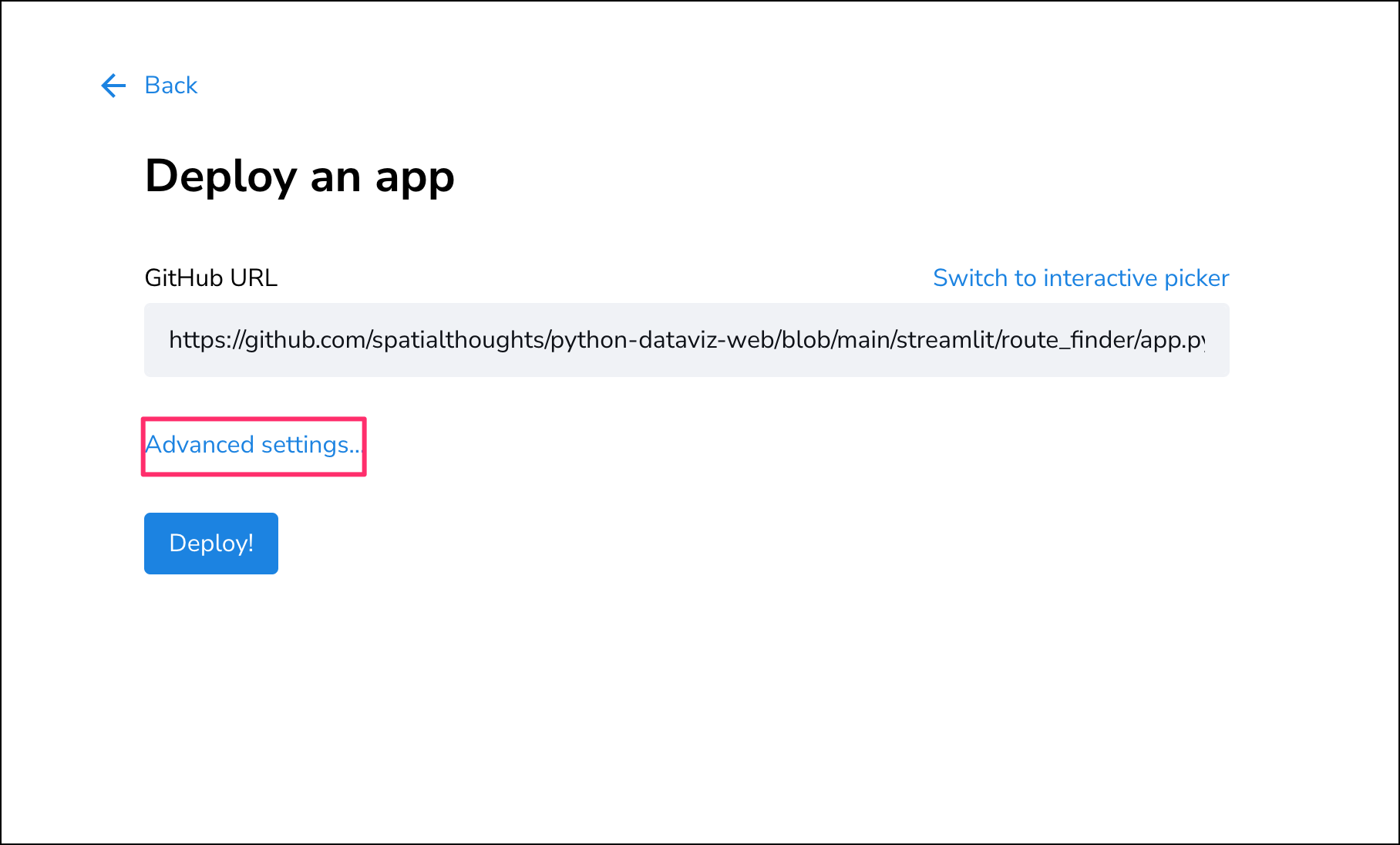1. This dialog allows you to store your private information required by your apps, such as API keys, username/password for your database, etc. For the Route Finder app, you need to enter the API Key for OpenRouteService. Visit OpenRouteService Dashboard and copy your API Key. Enter your API key in the following format and replace with your actual API key. Click Save.
'ORS_API_KEY' = '<your key>'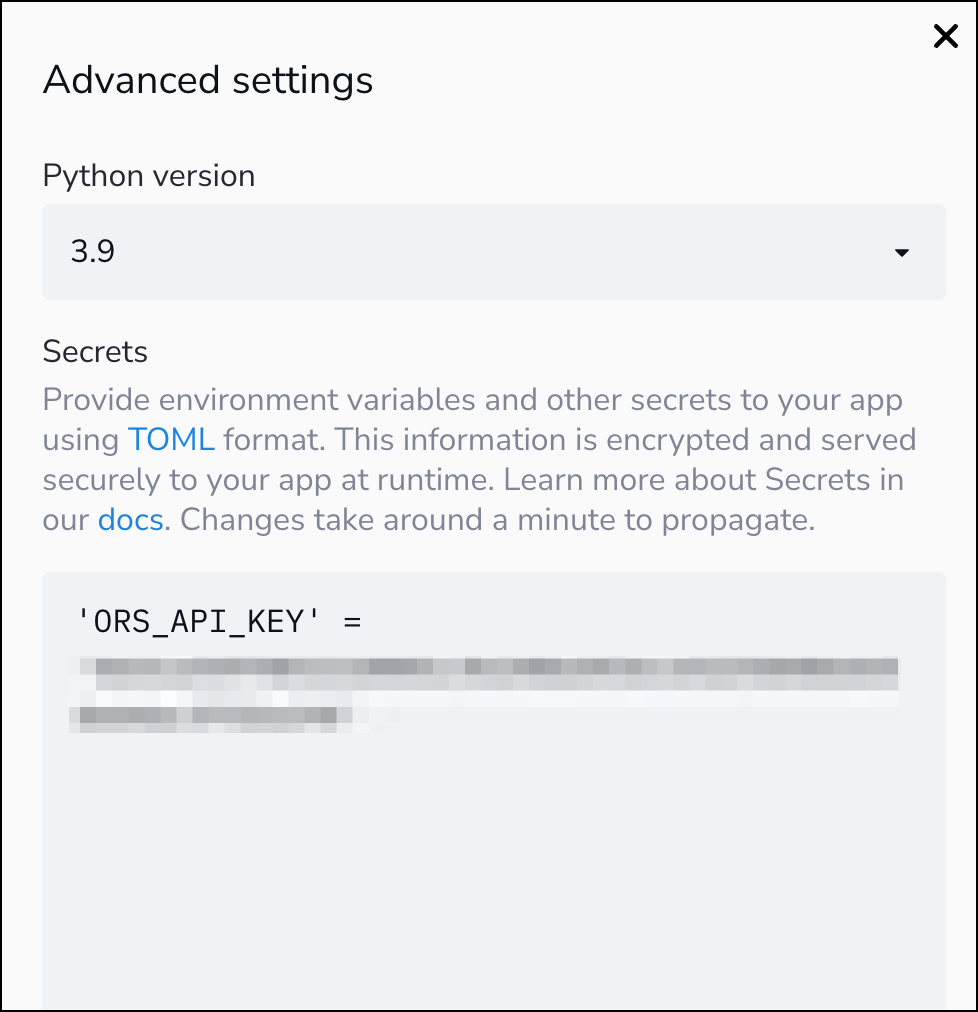1. You are now ready to deploy the app. Click Deploy!.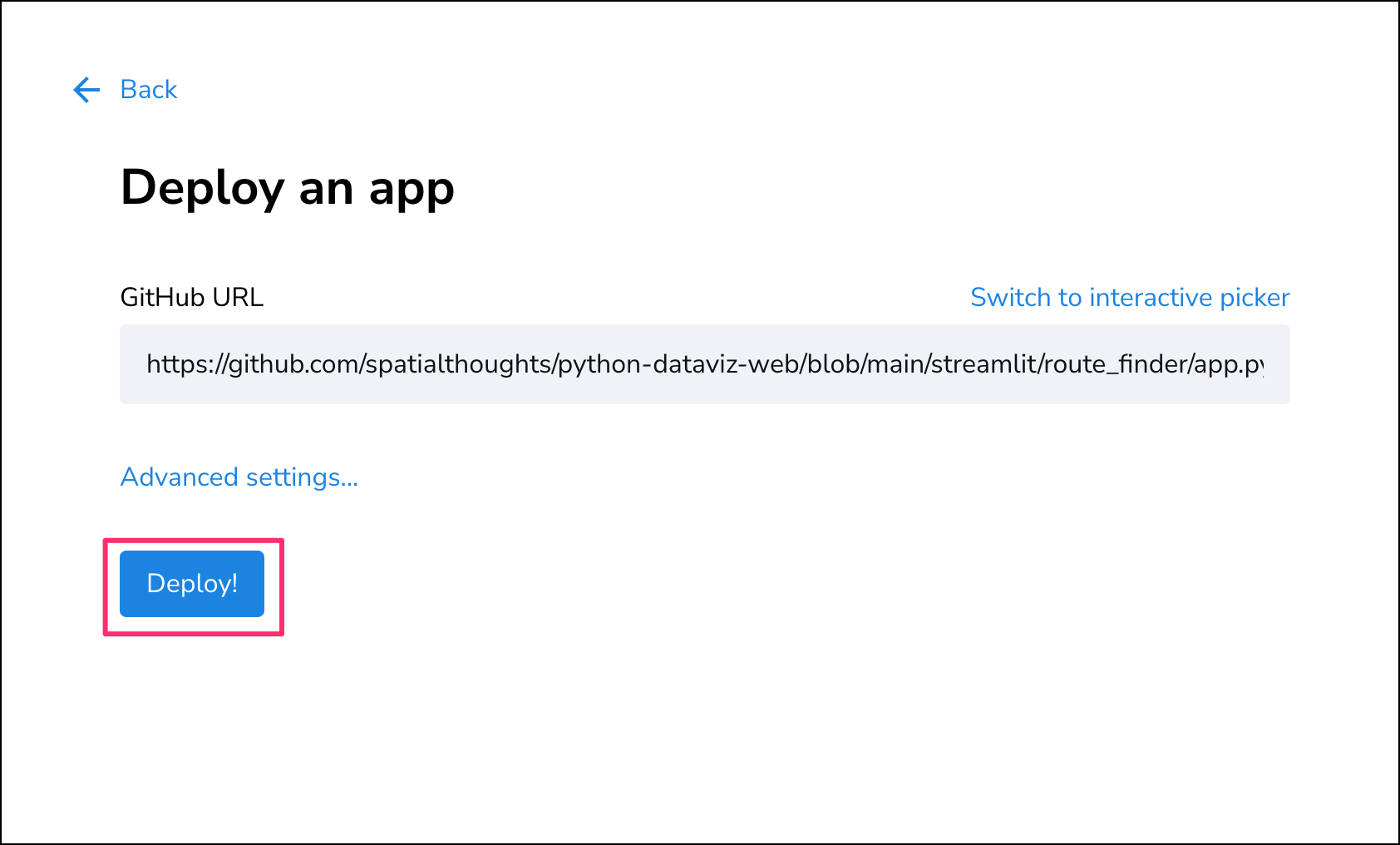1. Your app will now be deployed and will be accessible via the provided URL. You can visit your Dashboard to manage the app once it is deployed.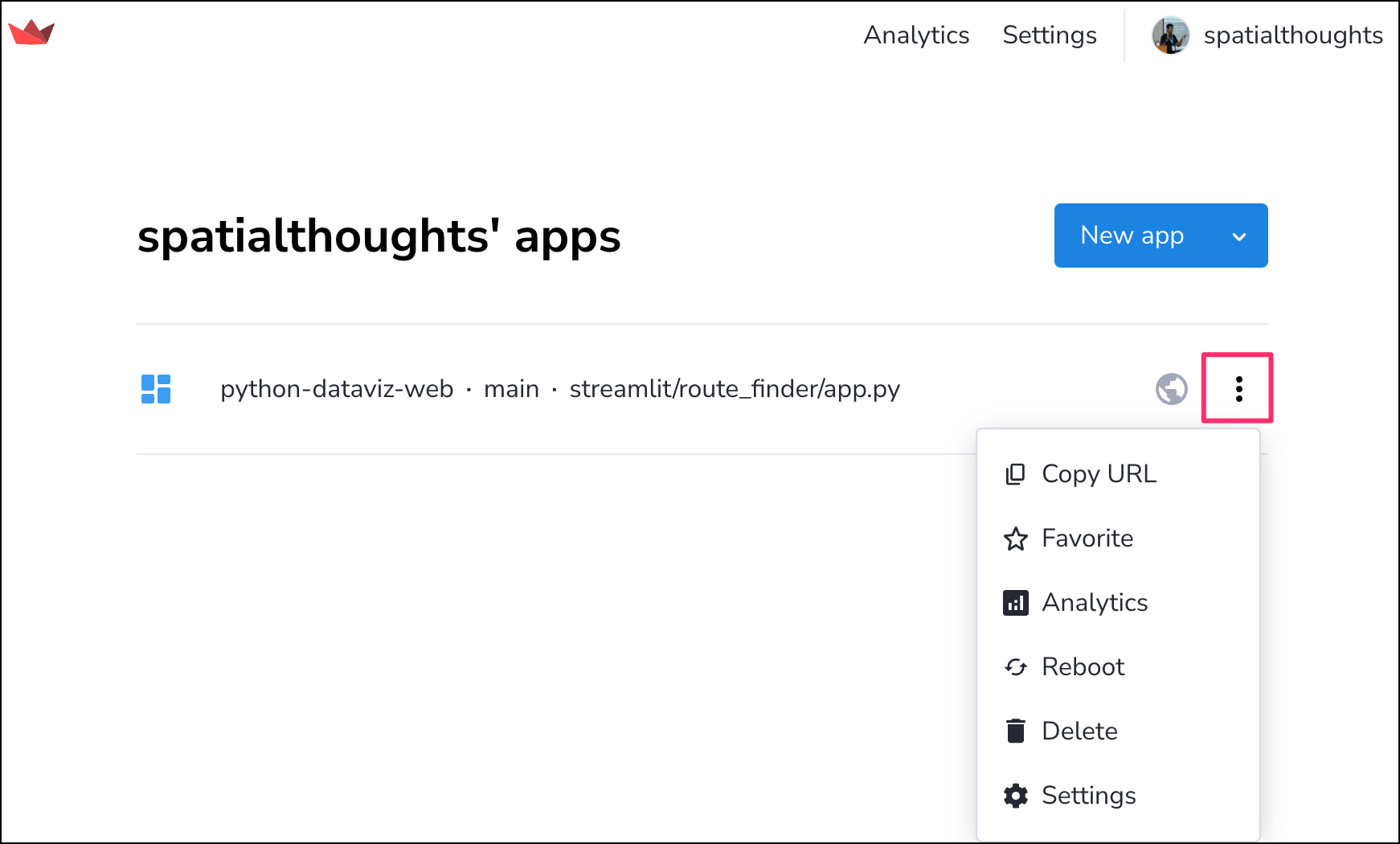The app is now live! Visit the Route Finder app to see it in action.

# Supplement

## Contextily### Creating an Artistic Rendering of a City

Contextily provides an easy way to render tiles for a location using the OpenStreetMap’s Nominatim API. Any location name from OpenStreetMap can be geocoded and displayed using the Contextily Place API.

We can use it to quickly create a high-resolution rendering of any city using any supported basemap with exact dimensions.

#### Setup

The following blocks of code will install the required packages and download the datasets to your Colab environment.

%%capture
!pip install contextily
import contextily as cx
from contextily import Place
import matplotlib.pyplot as plt
import os
data_folder = 'data'
output_folder = 'output'

if not os.path.exists(data_folder):
os.mkdir(data_folder)
if not os.path.exists(output_folder):
os.mkdir(output_folder)

#### Procedure

Replace the place_name below with the name of your chosen city, region or neighborhood and run it to query for its coordinates and bounding box for OpenStreetMap. If your query fails, you can visit OpenStreetMap and search for it to find the exact spelling of the place.

place_name = 'Bangalore'
place = Place(place_name, zoom=13)
fig, ax = plt.subplots(1, 1)
place.plot(ax=ax)
ax.set_axis_off()
plt.tight_layout()
plt.show()

You can choose from over 200 basemap styles created by different providers. Check the available styles using contextily.providers.

providers = cx.providers
providers

Let’s try the Stamen.Toner style. Some basemaps are available only upto a certain zoom level. If you get an error adjust the zoom level as well.

source = cx.providers.Stamen.Toner
zoom = 13
place = Place(place_name, zoom=zoom, source=source)

fig, ax = plt.subplots(1, 1)
place.plot(ax=ax)
ax.set_axis_off()
plt.tight_layout()
plt.show()

The Place API returns the rendering of the city based on its boundng box.

place = Place(place_name)
x_min, x_max, y_min, y_max = place.bbox_map
print('Original BBOX', x_min, x_max, y_min, y_max)

If we wanted to create a rendering for exact dimensions, we have to adjust the default bounding box. Here we want to create a rendering that will fit exactly to the chosen paper size. We compute the required ratio and adjust the bounds so the resulting ratio matches our paper size.

# Here we are using A4 paper size in Landscape orientation
# Swap width and height for Portrait orientation
# or choose any other dimensions
paper_width = 11.69
paper_height = 8.27

ratio = paper_width/paper_height

x_size = x_max - x_min
y_size = y_max - y_min

if ratio > 0:
x_size_required = ratio*(y_max - y_min)
difference =  x_size_required - x_size
x_min = x_min - difference/2
x_max = x_max + difference/2
else:
y_size_required = ratio*(x_max - x_min)
difference =  y_size_required - y_size
y_min = y_min - difference/2
y_max = y_max + difference/2
print('Adjusted BBOX', x_min, x_max, y_min, y_max)

Now we have the bounding box cooridnates, we can use the bounds2img method to fetch the tiles and create a map. The final rendering is saved as a PNG file in the Colab localstorage. You can open the Files tab from the left-hand panel in Colab and browse to the output folder. Locate the basemap.png file and click the button and select Download to download the file locally.

fig, ax = plt.subplots(1, 1)
fig.set_size_inches(paper_width, paper_height)

basemap, extent = cx.bounds2img(x_min, y_min, x_max, y_max, zoom=zoom, source=source)
ax.imshow(basemap, extent=extent)

ax.set_xlim(x_min, x_max)
ax.set_ylim(y_min, y_max)

ax.set_xticks([])
ax.set_yticks([])

output_file = 'basemap.png'
output_path = os.path.join(output_folder, output_file)
plt.show()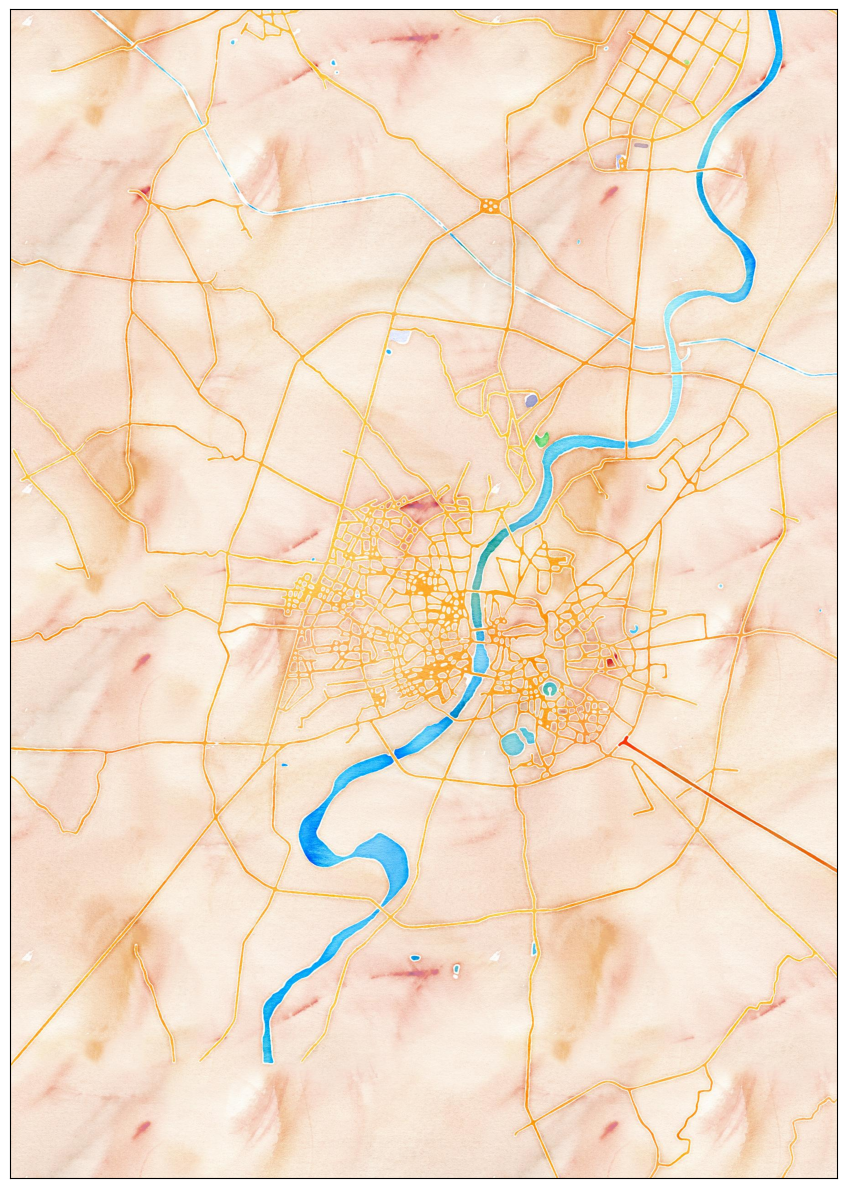### Elevation Profile Plot from a GPS Track

We will take a GPS track recorded from Strava app and create an elevation profile plot.

The following blocks of code will install the required packages and download the datasets to your Colab environment.

%%capture
!apt install libspatialindex-dev
!pip install fiona shapely pyproj rtree mapclassify
!pip install geopandas
import geopandas as gpd
import matplotlib.pyplot as plt
import matplotlib.dates as mdates
import os
import pandas as pd
data_folder = 'data'
output_folder = 'output'

if not os.path.exists(data_folder):
os.mkdir(data_folder)
if not os.path.exists(output_folder):
os.mkdir(output_folder)
def download(url):
filename = os.path.join(data_folder, os.path.basename(url))
if not os.path.exists(filename):
from urllib.request import urlretrieve
local, _ = urlretrieve(url, filename)
print('Downloaded ' + local)
filename = 'summit.gpx'
data_url = 'https://github.com/spatialthoughts/python-dataviz-web/raw/main/data/gps/'

download(data_url + filename)

#### Data Pre-Processing

gpx_path = os.path.join(data_folder, filename)
# GPX files contain many layers. Read the 'track_points' layer
gdf = gdf[['track_fid','ele', 'time', 'geometry']]
gdf

Let’s use the timestamp contained in the ‘time’ column as the index. This will allow us to filter and plot the time-series data easily. We must first convert the time column to datetime type with an appropriate timezone.

gdf['time'] = pd.to_datetime(gdf['time'])
gdf = gdf.set_index('time')
gdf.index = gdf.index.tz_convert('Asia/Kolkata')
gdf

Using time as index allows us to filter the data as follows

gdf_subset = gdf['2022-04-04T06:15:00':'2022-04-04T10:45:00']
gdf_subset

We can now plot the elevation against the timestamp using Matplotlib. Since we have a large number of timestamps, we can use set_major_locator() to define what labels will be present on the axis.

Since our timestamps are timezone aware, we can also plot a timezone name (i.e. IST) along with the label.

We can also use a different style for our plot. You can run plt.style.available to see all available styles.

plt.style.use('ggplot')
fig, ax = plt.subplots(1, 1)
fig.set_size_inches(15,7)
gdf_subset['ele'].plot(kind='line', ax=ax, color='#2b8cbe')
plt.tight_layout()
plt.title('Elevation Profile', fontsize = 18)
plt.ylabel('Elevation (meters)', size = 15)
plt.xlabel(None)
# Show a tick every 30 minute
xlocator = mdates.MinuteLocator(interval=30)

xformat = mdates.DateFormatter('%H:%M %Z', tz=gdf.index.tz)  # adds some extra formatting, but not required

ax.xaxis.set_major_locator(xlocator)
ax.xaxis.set_major_formatter(xformat)
ax.set_ylim([3200, 3700])
ax.fill_between(gdf.index, gdf['ele'].values, color='grey', alpha=0.3)
plt.show()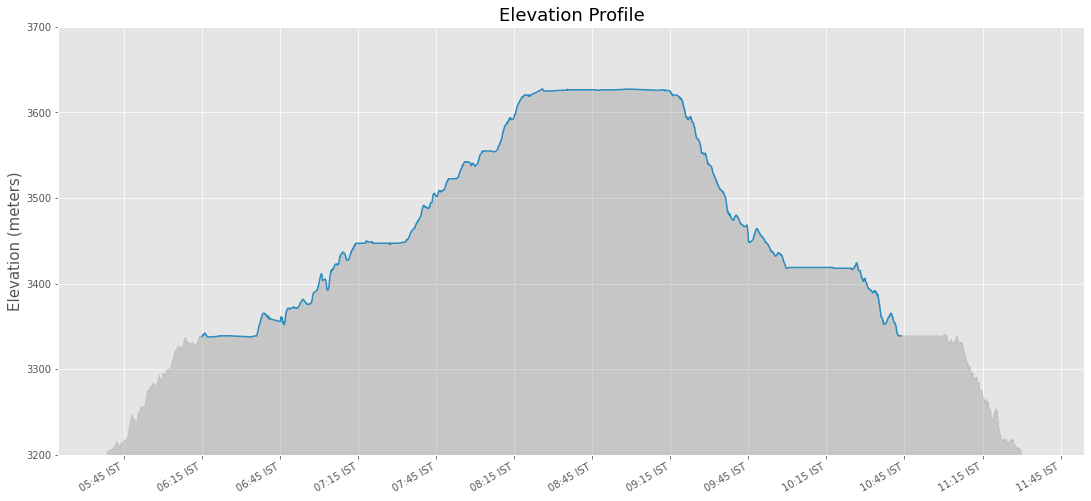plt.style.use('default')### Creating A Stacked Bar Chart

This example shows how to create a stacked chart with information about crime type in each bar. This visualization can plot 2 variables in a single plot.

The following blocks of code will install the required packages and download the datasets to your Colab environment.

import pandas as pd
import os
import matplotlib.pyplot as plt
data_folder = 'data'
output_folder = 'output'

if not os.path.exists(data_folder):
os.mkdir(data_folder)
if not os.path.exists(output_folder):
os.mkdir(output_folder)
def download(url):
filename = os.path.join(data_folder, os.path.basename(url))
if not os.path.exists(filename):
from urllib.request import urlretrieve
local, _ = urlretrieve(url, filename)
print('Downloaded ' + local)

We have 12 different CSV files containing crime data for each month of 2020. We download each of them to the data folder.

files = [
'2020-01-metropolitan-street.csv',
'2020-02-metropolitan-street.csv',
'2020-03-metropolitan-street.csv',
'2020-04-metropolitan-street.csv',
'2020-05-metropolitan-street.csv',
'2020-06-metropolitan-street.csv',
'2020-07-metropolitan-street.csv',
'2020-08-metropolitan-street.csv',
'2020-09-metropolitan-street.csv',
'2020-10-metropolitan-street.csv',
'2020-11-metropolitan-street.csv',
'2020-12-metropolitan-street.csv'
]

data_url = 'https://github.com/spatialthoughts/python-dataviz-web/raw/main/data/crime/'

for f in files:
url = os.path.join(data_url + f)
download(url)

#### Data Pre-Processing

It will be helpful to merge all 12 CSV files into a single dataframe. We can use pd.concat() to merge a list of dataframes.

dataframe_list = []

for f in files:
filepath = os.path.join(data_folder, f)
dataframe_list.append(df)

merged_df = pd.concat(dataframe_list)
counts_by_type = merged_df.groupby(['Month', 'Crime type']).size()
counts_by_type

The result is not in a suitable format for plotting. We call unstack() to create a dataframe.

counts_df = counts_by_type.unstack()
counts_df

#### Creating a Chart

Now we can create the stacked bar chart. Instead of the default legend, we create a horizontal legend with a frame using the legend() function.

fig, ax = plt.subplots(1, 1)
fig.set_size_inches(20,10)
counts_df.plot(kind='bar', stacked=True, ax=ax, colormap='tab20')
ax.legend(loc='upper center', ncol=5, frameon=True, bbox_to_anchor=(0.5, 1.1), fancybox=True, shadow=True)
ax.spines['right'].set_visible(False)
ax.spines['top'].set_visible(False)
ax.set_xlabel('Year', size = 15)
ax.set_ylabel('Number of Incidents', size = 15)
ax.set_title('Crime in London (2020)', size = 18, y=1.1)
output_folder = 'output'
output_path = os.path.join(output_folder, 'stacked_chart.jpg')
plt.savefig(output_path)
plt.show()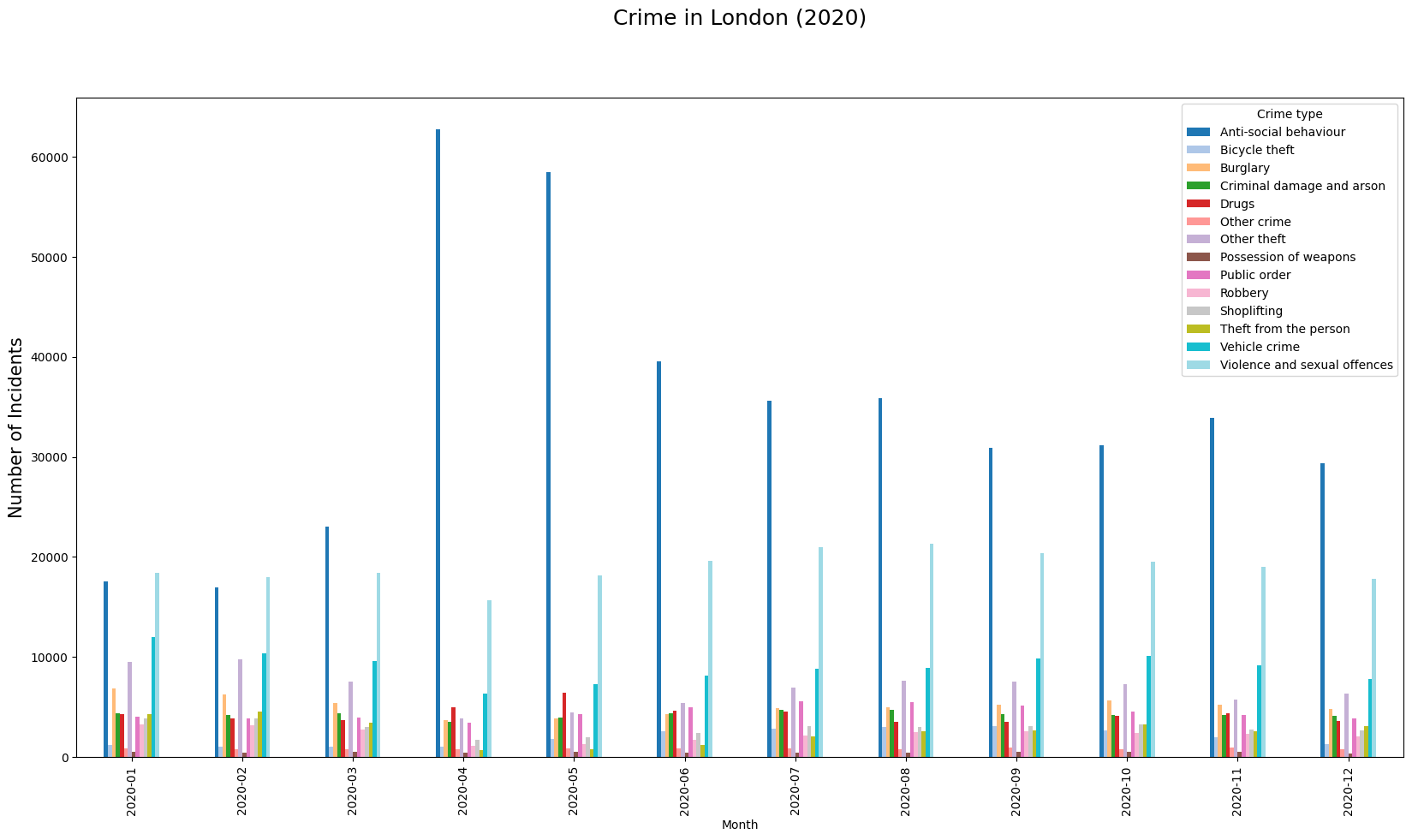### Labeling Features

This example shows how to add labels to vector layer features using Matplotlib. We will take a polygon layer of districts and add an annotation to show the district name at the centroid of each polygon.

%%capture
!apt install libspatialindex-dev
!pip install fiona shapely pyproj rtree mapclassify
!pip install geopandas
import os
import geopandas as gpd
import matplotlib.pyplot as plt
data_folder = 'data'
output_folder = 'output'

if not os.path.exists(data_folder):
os.mkdir(data_folder)
if not os.path.exists(output_folder):
os.mkdir(output_folder)
def download(url):
filename = os.path.join(data_folder, os.path.basename(url))
if not os.path.exists(filename):
from urllib.request import urlretrieve
local, _ = urlretrieve(url, filename)

filename = 'karnataka.gpkg'
data_url = 'https://github.com/spatialthoughts/python-dataviz-web/raw/main/data/osm/'
download(data_url + filename)
Downloaded data/karnataka.gpkg

#### Data Pre-Processing

We read the districts layer from the input geopackage using GeoPandas.

path = os.path.join(data_folder, filename)
districts_gdf = gpd.read_file(path, layer='karnataka_districts')

Let’s say we want to add a label for each of the distrit polygons. First, we need to decide the anchor position of the label. We can use representative_point() to get a point inside each polygon that best represents the geometry. It is similar to a centroid, but is guranteed to be inside the polygon. Below code creates a new field in the GeoDataFrame called label_position with the coordinates of the anchor point.

def get_label_position(row):
geometry = row['geometry']
location = geometry.representative_point()
# We need the location as a tuple of x,y coordinates
location_coords = location.coords
return location_coords

districts_gdf['label_position'] = districts_gdf.apply(get_label_position, axis=1)
districts_gdf

#### Annotating Labels

We can now plot the districts and add an annotation for each polygon. We iterate over each row of the GeoDataFrame and add the annotation with name of the district at its centroid coordinates using annotate() function.

fig, ax = plt.subplots(1, 1)
fig.set_size_inches(10,15)
districts_gdf.plot(ax=ax, linewidth=1, facecolor='none', edgecolor='#252525')

for idx, row in districts_gdf.iterrows():
ax.annotate(text=row['DISTRICT'], xy=row['label_position'], horizontalalignment='center')
ax.set_axis_off()
plt.tight_layout()
plt.show()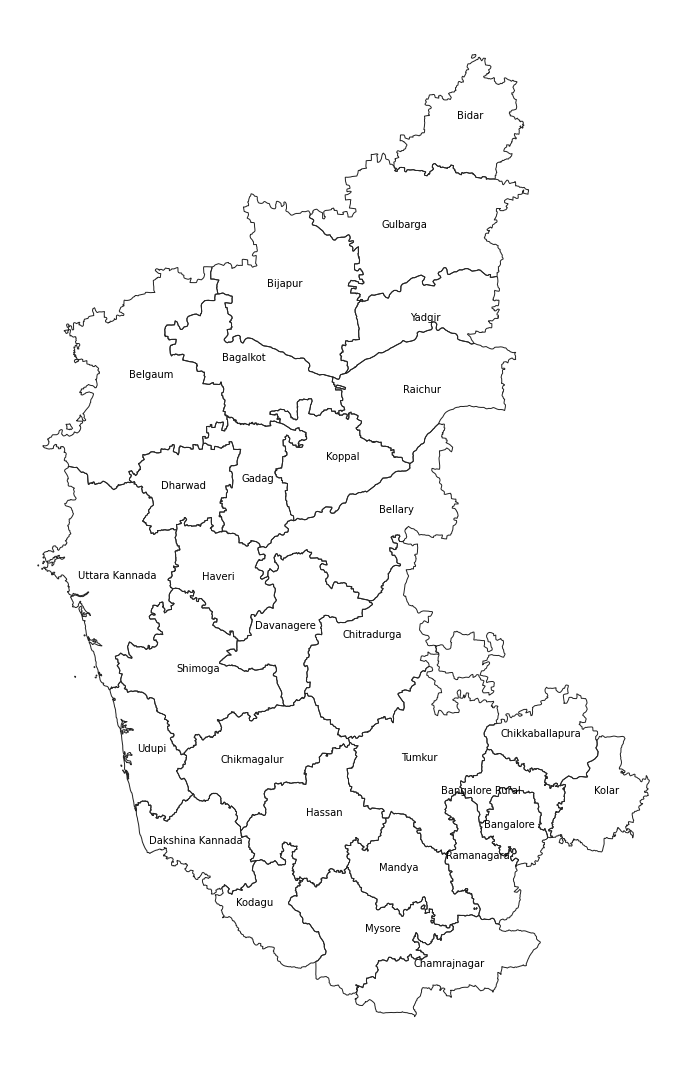### Creating Time-Series Charts

import pandas as pd
import os
import matplotlib.pyplot as plt
import matplotlib.dates as mdates
data_folder = 'data'
output_folder = 'output'

if not os.path.exists(data_folder):
os.mkdir(data_folder)
if not os.path.exists(output_folder):
os.mkdir(output_folder)
def download(url):
filename = os.path.join(data_folder, os.path.basename(url))
if not os.path.exists(filename):
from urllib.request import urlretrieve
local, _ = urlretrieve(url, filename)

data_url = 'https://github.com/spatialthoughts/python-dataviz-web/raw/main/data/misc/'
filename = 'ndvi_data.xlsx'
download(data_url + filename)

#### Data Pre-Processing

filepath = os.path.join(data_folder, filename)
df

We set the ‘Date’ column as the index of the dateframe. This will allow us to filter and plot the time-series data easily.

df = df.set_index(pd.to_datetime(df['Date']))
df

Create a chart with time-series of values in the ‘NDVI’ column. We use mdates module to control the tick-marks on X-Axis.

fig, ax = plt.subplots(1, 1)
fig.set_size_inches(20,10)
df.plot(y='NDVI', kind='line', ax=ax,
marker='o', markersize=2, color='#238b45',
label='Original NDVI')

ax.xaxis.set_major_locator(mdates.MonthLocator(interval=6))
ax.xaxis.set_major_formatter(mdates.DateFormatter("%Y-%m"))

ax.grid('on')
ax.set_title('NDVI Time-Series')
ax.set_ylabel('NDVI')

# Save the plot
output_folder = 'output'
output_path = os.path.join(output_folder, 'ndvi_time_series.png')
plt.savefig(output_path)

plt.show()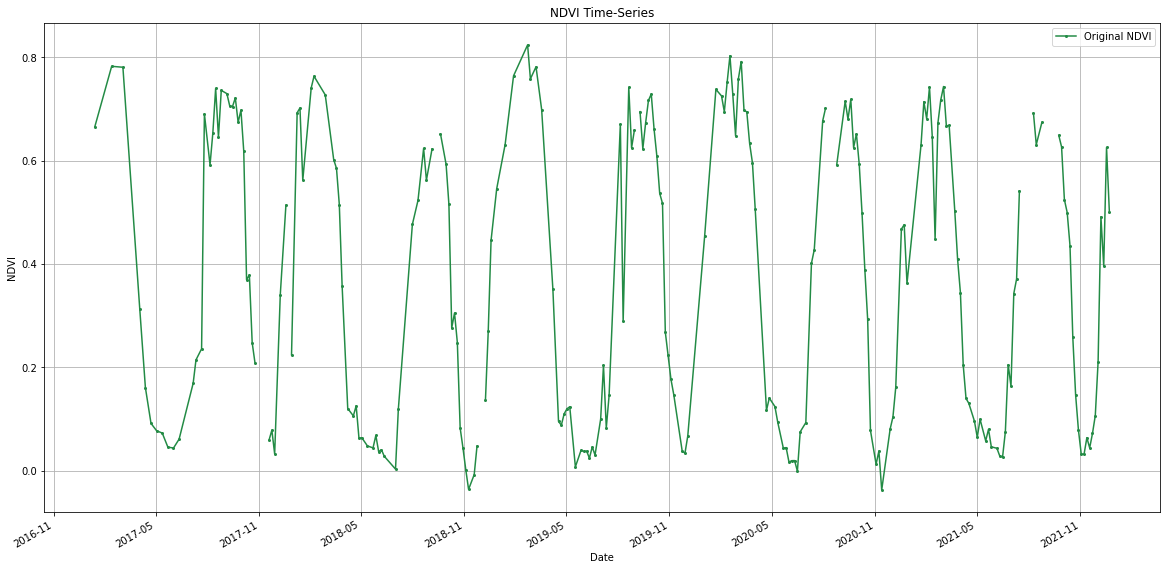#### Time Series Smoothing with Moving Average

Pandas has built-in method rolling() to allow us to compute moving averages. Let’s smooth the time-series with a moving-window average.

window_size_days = 30
window = '{}D'.format(window_size_days)
df_smooth = df.copy()
df_smooth['NDVI_smooth'] = df_smooth['NDVI'].rolling(window, center=True).mean()
df_smooth
fig, ax = plt.subplots(1, 1)
fig.set_size_inches(20,10)
df_smooth.plot(y='NDVI', kind='line', ax=ax,
marker='o', markersize=1, color='#66c2a4', linestyle='dotted',
label='Original')
df_smooth.plot(y='NDVI_smooth', kind='line', ax=ax,
marker='o', linewidth= 2, markersize=0, color='#238b45',
label='{} Day Moving-Average'.format(window_size_days))
ax.xaxis.set_major_locator(mdates.MonthLocator(interval=6))
ax.xaxis.set_major_formatter(mdates.DateFormatter("%Y-%m"))

ax.grid('on')

ax.set_title('NDVI Time-Series')
ax.set_ylabel('NDVI')

# Save the plot
output_folder = 'output'
output_path = os.path.join(output_folder, 'smooth_ndvi_time_series.png')
plt.savefig(output_path)

plt.show()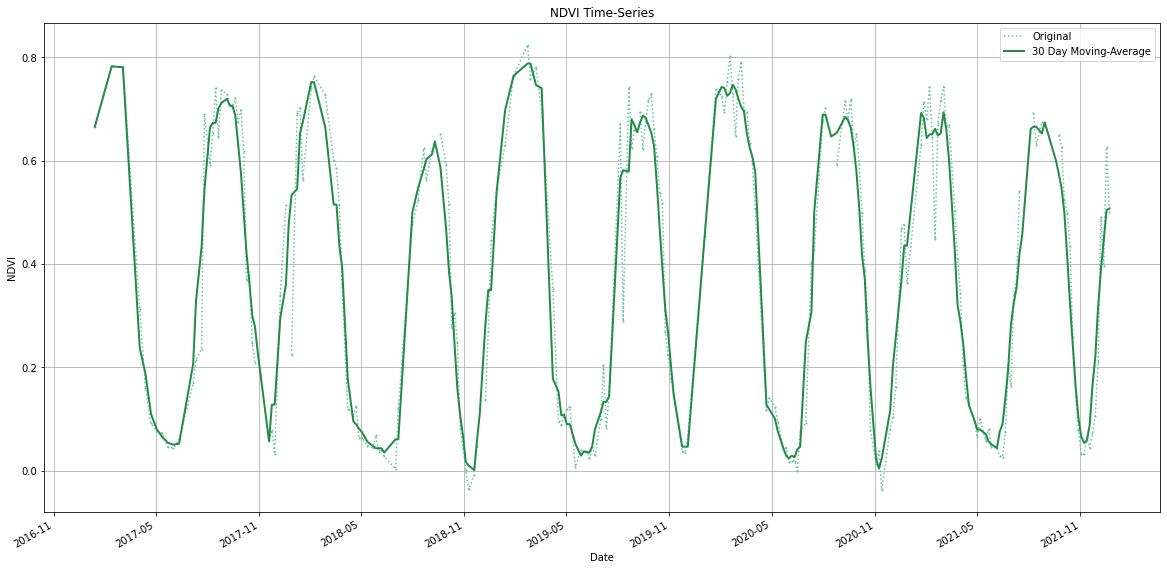### Feature Correlation Matrix

Correlation Matrix is used in Machine Learning to identify redudant features that are correlated. We will take a table of feature samples generated from a multiband image and create a correlation matrix. This matrix is used to identify and visualize patterns in the given data and select features for a machine learning model.

The following blocks of code will install the required packages and download the datasets to your Colab environment.

import matplotlib.pyplot as plt
import os
import pandas as pd
import numpy as np
data_folder = 'data'
output_folder = 'output'

if not os.path.exists(data_folder):
os.mkdir(data_folder)
if not os.path.exists(output_folder):
os.mkdir(output_folder)
def download(url):
filename = os.path.join(data_folder, os.path.basename(url))
if not os.path.exists(filename):
from urllib.request import urlretrieve
local, _ = urlretrieve(url, filename)

data_url = 'https://github.com/spatialthoughts/python-dataviz-web/raw/main/data/misc/'
csv_name = 'feature_sample_data.csv'


Downloaded data/feature_sample_data.csv

#### Data Pre-Processing

csv_path = os.path.join(data_folder, csv_name)
table = pd.read_csv(csv_path)

We use Pandas’ pd.DataFrame.corr() method to calculate pairwise Pearson’s Correlation Coefficient for each variable.

correlations = table.corr()

#### Plotting using Matplotlib

We can use Matplotlib’s matplotlib.pyplot.matshow to display any array as a matrix.

fig, ax = plt.subplots(1, 1)
fig.set_size_inches(18,18)
im = ax.matshow(correlations, vmin=-1, vmax=1)
fig.colorbar(im, shrink=0.8)

ticks = np.arange(0,len(table.columns),1)
ax.set_xticks(ticks)
ax.set_yticks(ticks)
ax.set_xticklabels(table.columns)
ax.set_yticklabels(table.columns)

output_folder = 'output'
output_path = os.path.join(output_folder, 'correlation_matrix.png')

plt.savefig(output_path, dpi=300)
plt.show()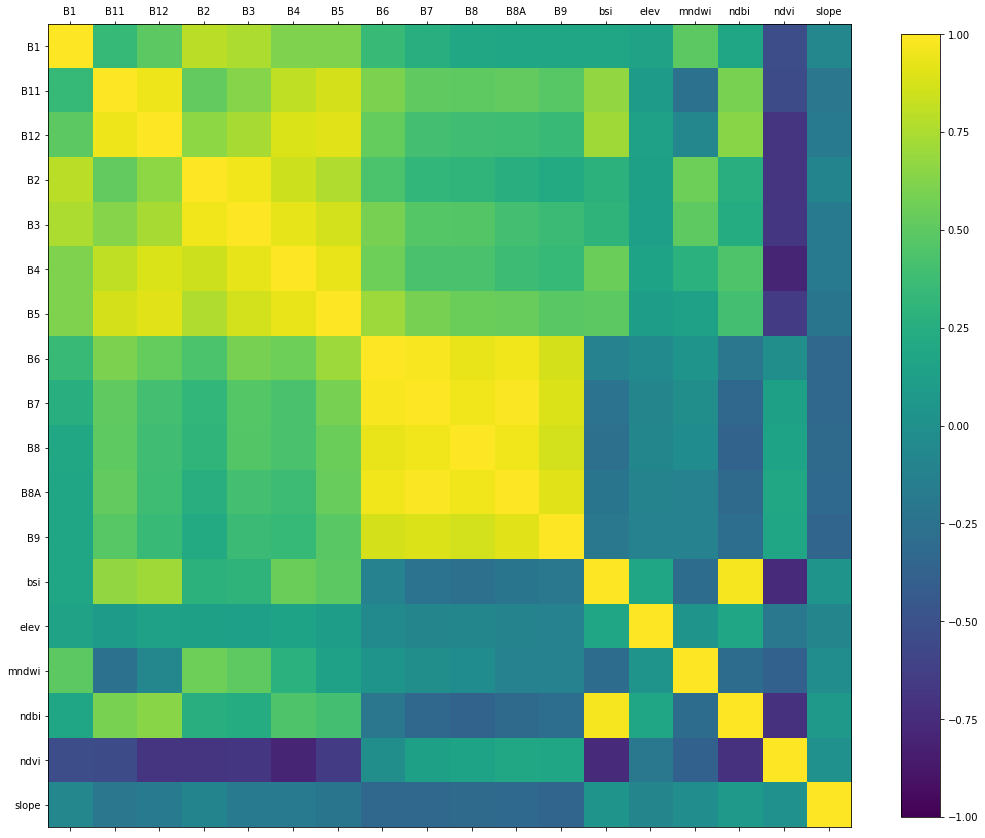## Animation### Creating Animated Maps

We take the dataset for 2017 solar eclipse and animate the path of the solar eclipse.

The following blocks of code will install the required packages and download the datasets to your Colab environment.

%%capture
!apt install libspatialindex-dev
!pip install fiona shapely pyproj rtree
!pip install geopandas
!pip install contextily
import contextily as cx
import geopandas as gpd
import os
import matplotlib.pyplot as plt
from matplotlib.animation import FuncAnimation, PillowWriter
data_folder = 'data'
output_folder = 'output'

if not os.path.exists(data_folder):
os.mkdir(data_folder)
if not os.path.exists(output_folder):
os.mkdir(output_folder)
def download(url):
filename = os.path.join(data_folder, os.path.basename(url))
if not os.path.exists(filename):
from urllib.request import urlretrieve
local, _ = urlretrieve(url, filename)

path_shapefile = 'upath17'
umbra_shapefile = 'umbra17'
shapefile_exts = ['.shp', '.shx', '.dbf', '.prj']
data_url = 'https://github.com/spatialthoughts/python-dataviz-web/raw/main/data/eclipse/'

for shapefile in [path_shapefile, umbra_shapefile]:
for ext in shapefile_exts:
url = data_url + shapefile + ext
download(url)

#### Data Pre-Processing

path_shapefile_path = os.path.join(data_folder, path_shapefile + '.shp')
path_gdf = gpd.read_file(path_shapefile_path)
umbra_shapefile_path = os.path.join(data_folder, umbra_shapefile + '.shp')
umbra_gdf = gpd.read_file(umbra_shapefile_path)
path_reprojected = path_gdf.to_crs('epsg:3857')
umbra_reprojected = umbra_gdf.to_crs('epsg:3857')
path_boundary = path_reprojected.geometry.unary_union
umbra_subset = umbra_reprojected[umbra_reprojected.geometry.intersects(path_boundary)]

#### Creating Animation

We use Matplotlibs FuncAnimation function from the animation module to create an animation with each frame showing the position of the umbra through the solar eclipse.

fig, ax = plt.subplots(1, 1)
fig.set_size_inches(15,7)
plt.tight_layout()

def animate(i):
ax.clear()
# Get the point from the points list at index i
umbra_filtered = umbra_subset.iloc[i:i+1]
path_reprojected.plot(ax=ax, facecolor='#cccccc', edgecolor='#969696', alpha=0.5)
umbra_filtered.plot(ax=ax, facecolor='#252525', edgecolor='#636363', alpha=0.5)
ax.set_axis_off()
props = dict(boxstyle='round', facecolor='wheat', alpha=0.5)
time = umbra_filtered.iloc.Time
text = 'Time: {} UTC'.format(time)
ax.text(0.05, 0.20, text, transform=ax.transAxes, fontsize=16,
verticalalignment='top', bbox=props)
ax.set_title('2017 Total Solar Eclipse Path', size = 18)

ani = FuncAnimation(fig, animate, frames=len(umbra_subset),
interval=500, repeat=True, cache_frame_data=True)
plt.close()
import matplotlib as mpl
mpl.rcParams['animation.embed_limit'] = 2**128
from IPython.display import HTML
HTML(ani.to_jshtml())
output_folder = 'output'
output_path = os.path.join(output_folder, 'solar_eclipse.gif')

ani.save(output_path, writer=PillowWriter(fps=5))

## LeafmapLeafmap comes with handy utilities to work with OpenStreetMap data. Using the popular package OSMNx in the background, it provides utility functions to download and visualize data from the OSM database.

%%capture
!apt install libspatialindex-dev
!pip install fiona shapely pyproj rtree mapclassify
!pip install geopandas
!pip install leafmap
!pip install osmnx
import os
import geopandas as gpd
import folium
import leafmap.foliumap as leafmap
import osmnx
data_folder = 'data'
output_folder = 'output'

if not os.path.exists(data_folder):
os.mkdir(data_folder)
if not os.path.exists(output_folder):
os.mkdir(output_folder)

We can easily download data for a city or a region by its name using the leafmap.osm_gdf_from_place() function. We can specify the list of required tags using a dictionary. See OSM Wiki for a complete list of tags and values.

You can also download data using a bounding box using leafmap.osm.osm_gdf_from_bbox() function.

Reference: leafmap.osm_gdf_from_place

parking_gdf = leafmap.osm_gdf_from_place(
'Bangalore',
tags={'amenity': ['parking', 'parking_space', 'parking_entrance']}
)

The GeoDataFrame has a hierarchical MultiIndex. Let’s flatten it using reset_index()

parking_gdf = parking_gdf.reset_index(level=[0,1])

The result has many columns. Let’s filter to required columns.

parking_gdf_subset = parking_gdf[['amenity','parking', 'access', 'geometry']]

The results contains both points and polygon features. Let’s separate them out.

parking_zones = parking_gdf_subset[
parking_gdf_subset['geometry'].apply(lambda x : x.type == 'Polygon' )]
parking_locations = parking_gdf_subset[
parking_gdf_subset['geometry'].apply(lambda x : x.type == 'Point' )]

We can save the resulting GeoDataFrame to a GeoPackage.

output_file = 'parking.gpkg'
output_path = os.path.join(output_folder, output_file)
parking_zones.to_file(driver='GPKG', filename=output_path, layer='zones')
parking_locations.to_file(driver='GPKG', filename=output_path, layer='locations')

### Visualizing OSM Data

The leafmap.osm module has many functions that can add OSM data directy to the map. Here we use add_osm_from_geocode() function to add the boundary of a region from OSM. In addition, we can select a basemap from leafmap.basemaps.keys() for the map.

m = leafmap.Map(width=800, height=500)
m

We can add the GeoDataFrame to the map as well using GeoPanda’s explore() function which allows us to customize the marker’s shape, size for the point layer.

m = leafmap.Map(width=800, height=500)
parking_zones.explore(
style_kwds={'fillOpacity': 0.3, 'weight': 0.5},
color='orange',
name='parking zones',
m=m)
parking_locations.explore(
marker_type='circle',
color='yellow',
name='parking locations',
m=m)
m`